# ML Aggarwal Solutions For Class 9 Maths Chapter 5 Simultaneous Linear Equations

ML Aggarwal Solutions For Class 9 Maths Chapter 5 Simultaneous Linear Equations gives a clear understanding of the concept of simultaneous linear equations. BYJU’S provides you solutions, which are designed by our subject experts. Students can easily download ML Aggarwal Solutions from our website in PDF format for free. This chapter deals with solving for two variables, when two equations are given. Solving different problems helps students to improve time management skills. It also increases their confidence, so that they can be stress-free during exams.

Two linear equations in two variables, taken together are called simultaneous linear equations. In ML Aggarwal solutions For Class 9 Maths Chapter 5, we come across solving the simultaneous linear equations. Sign in BYJU’S to get more practice on these solutions.

## Download the Pdf of ML Aggarwal Solutions for Class 9 Maths Chapter 5- Simultaneous Linear Equations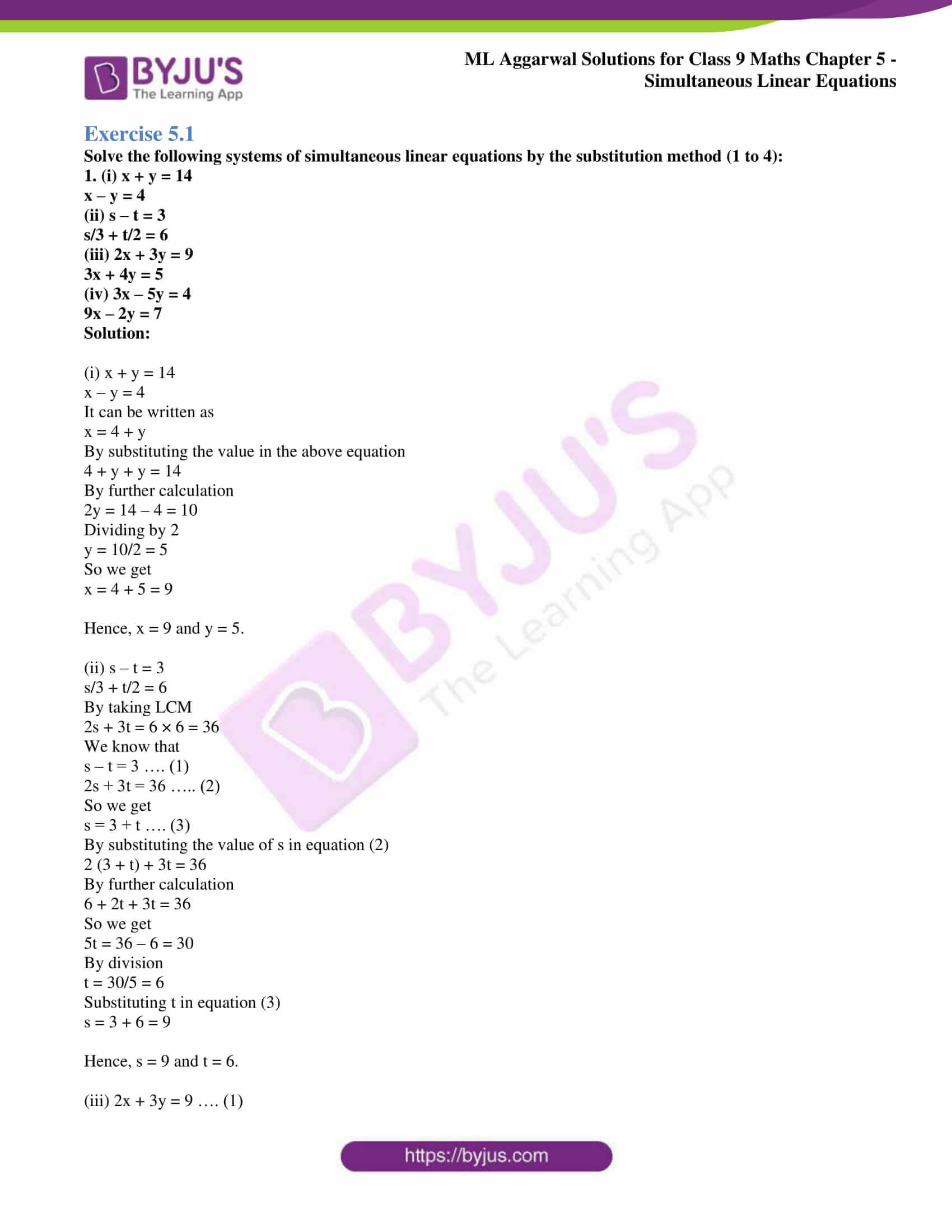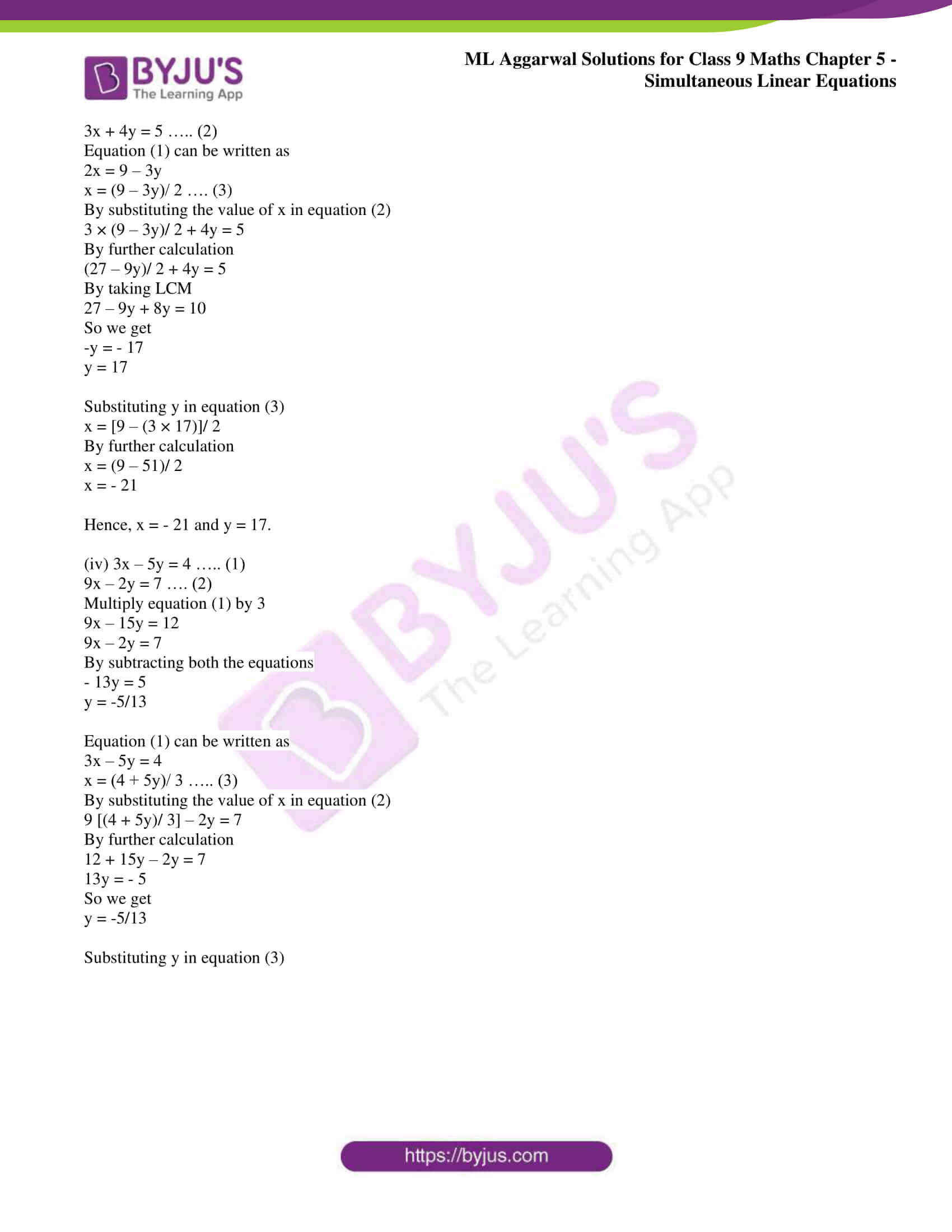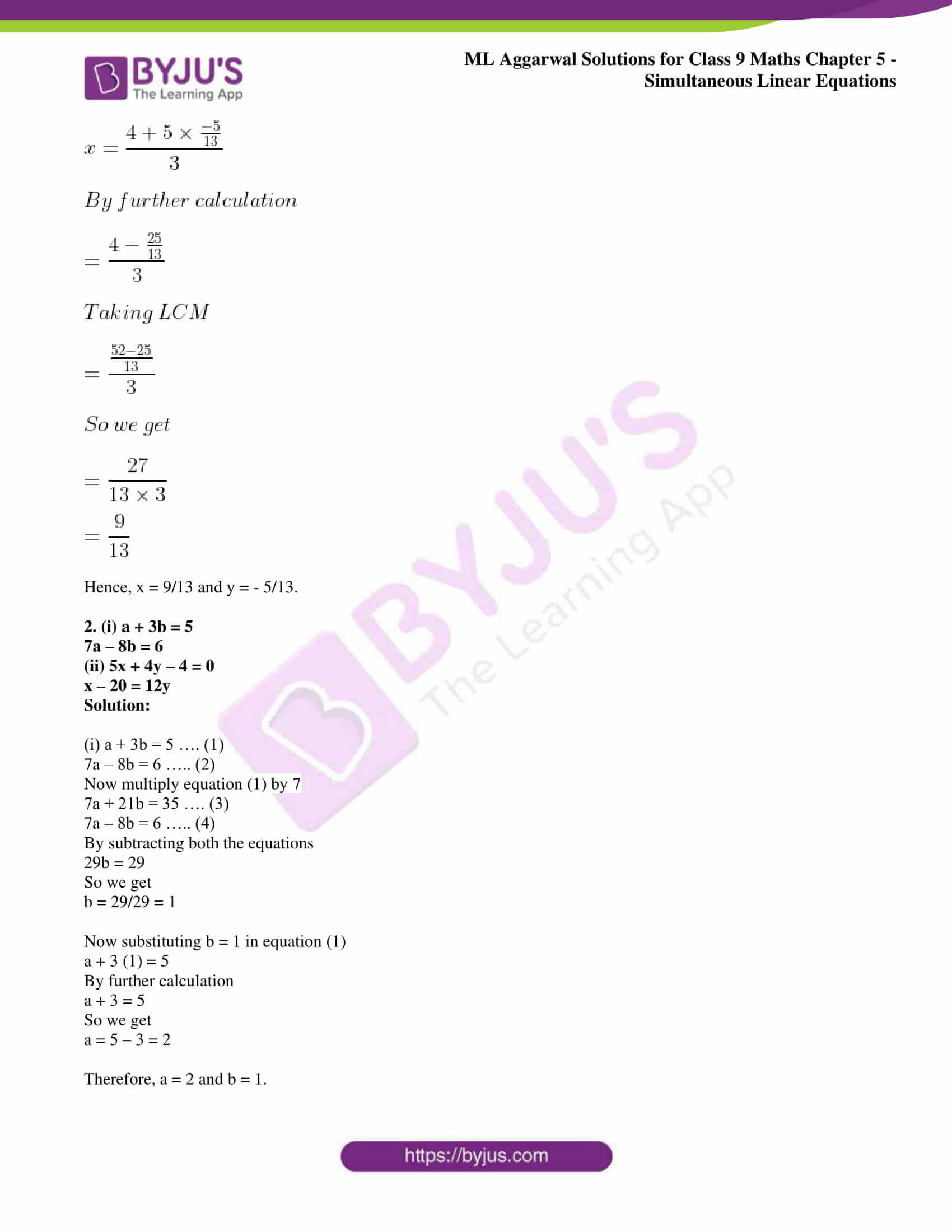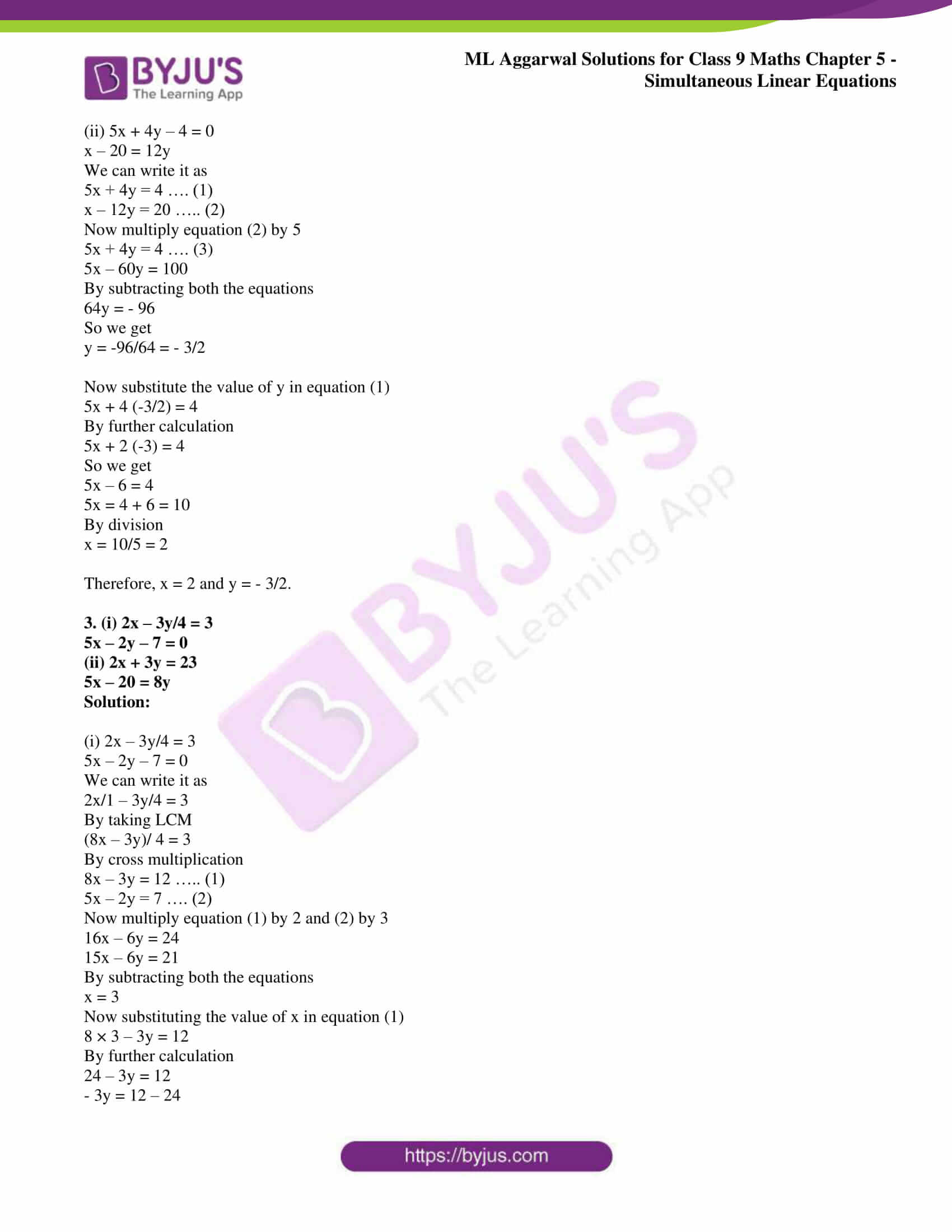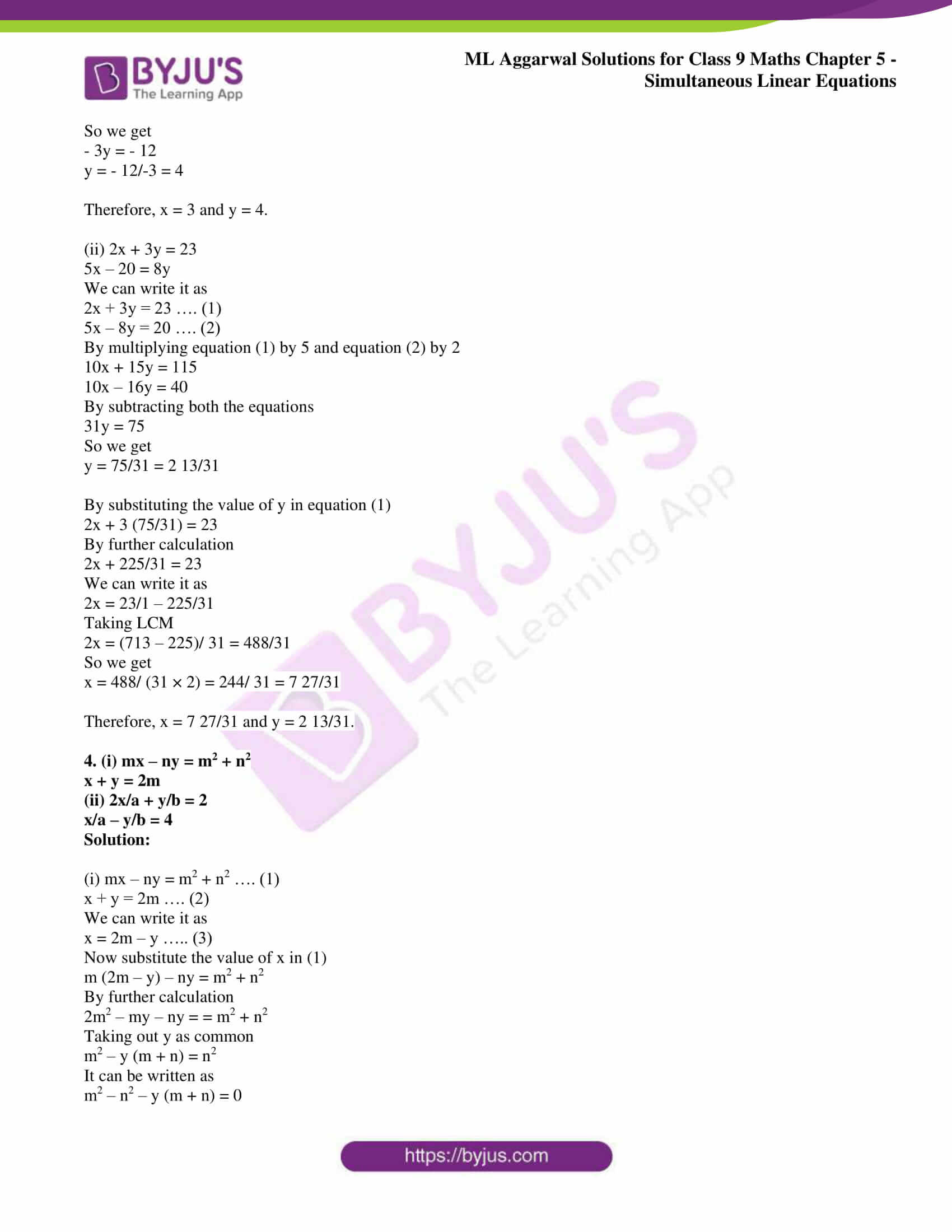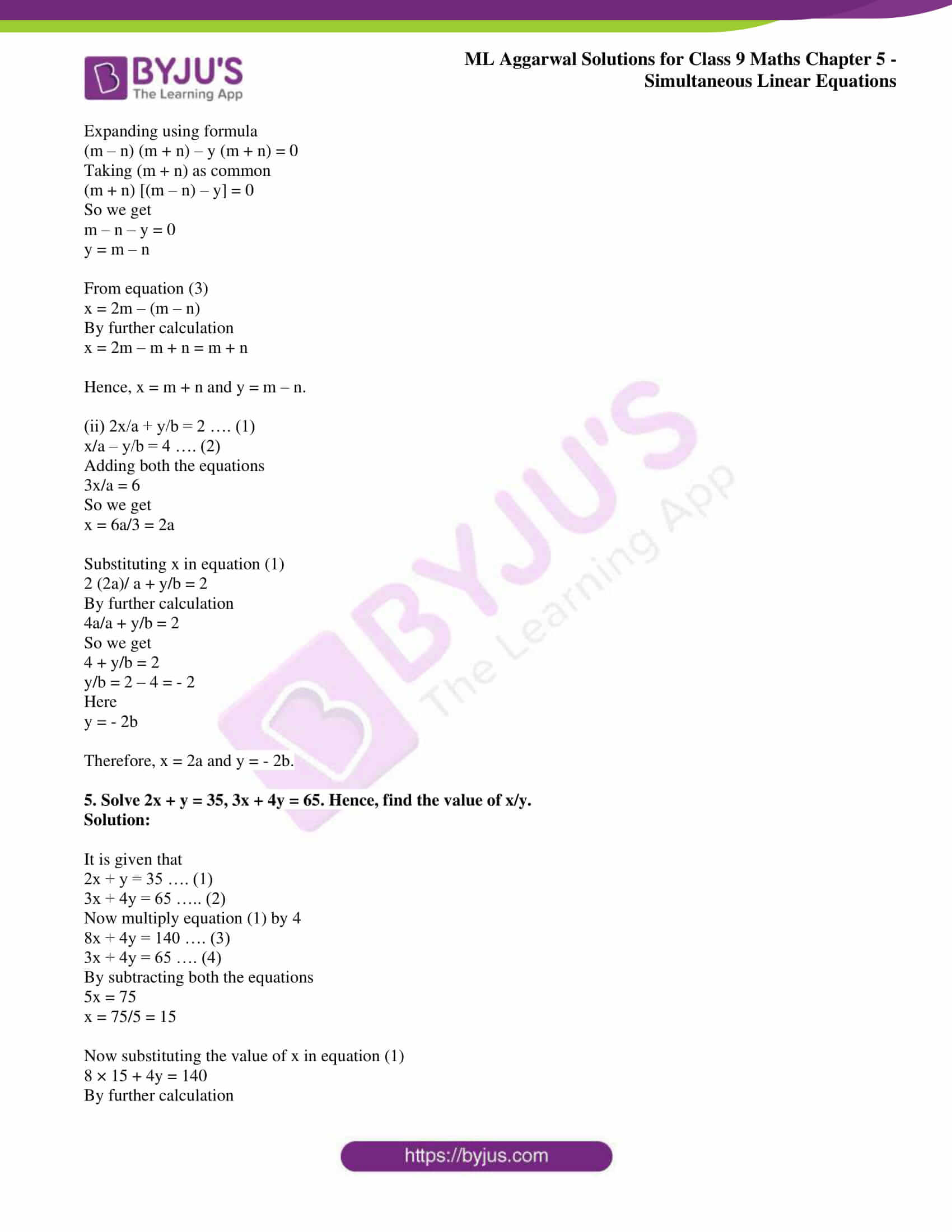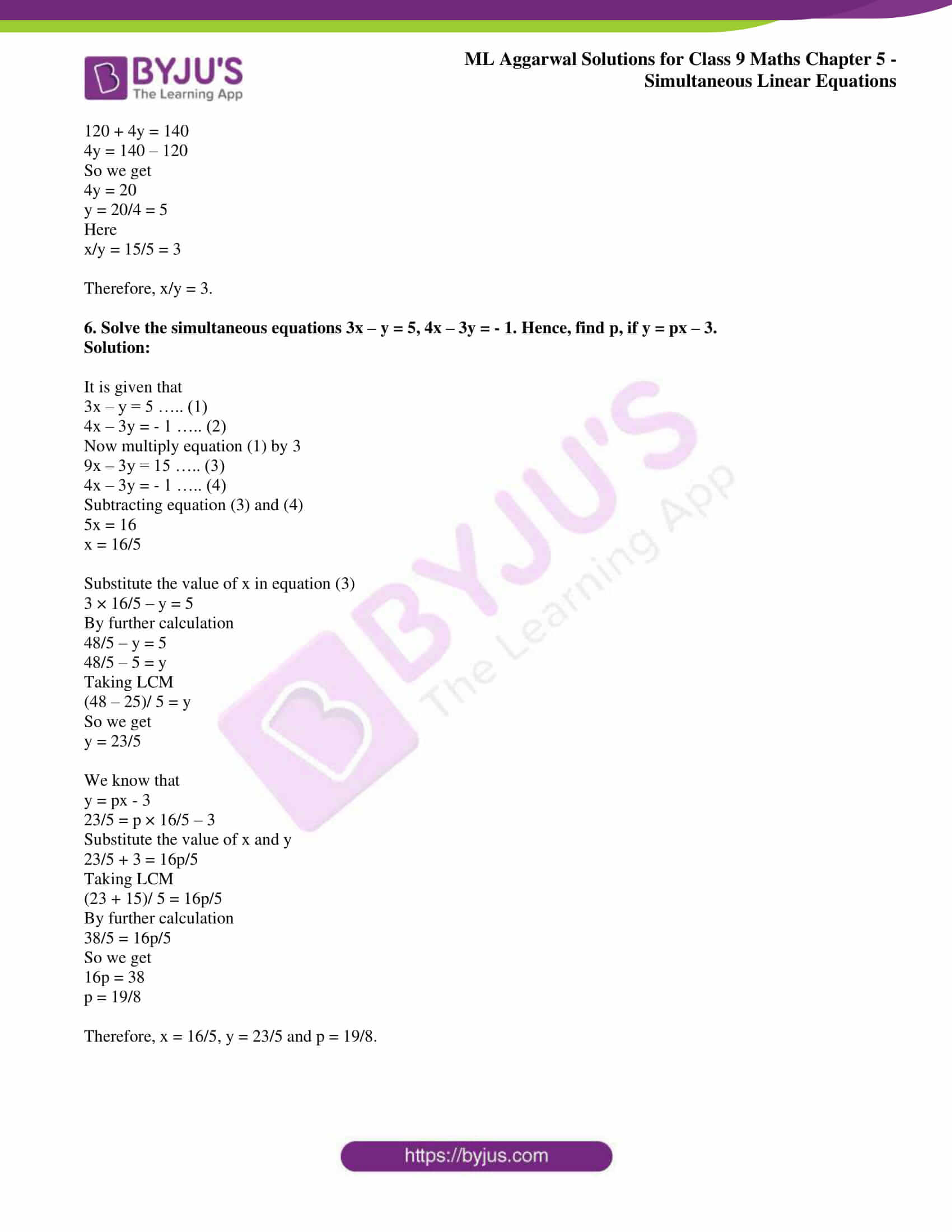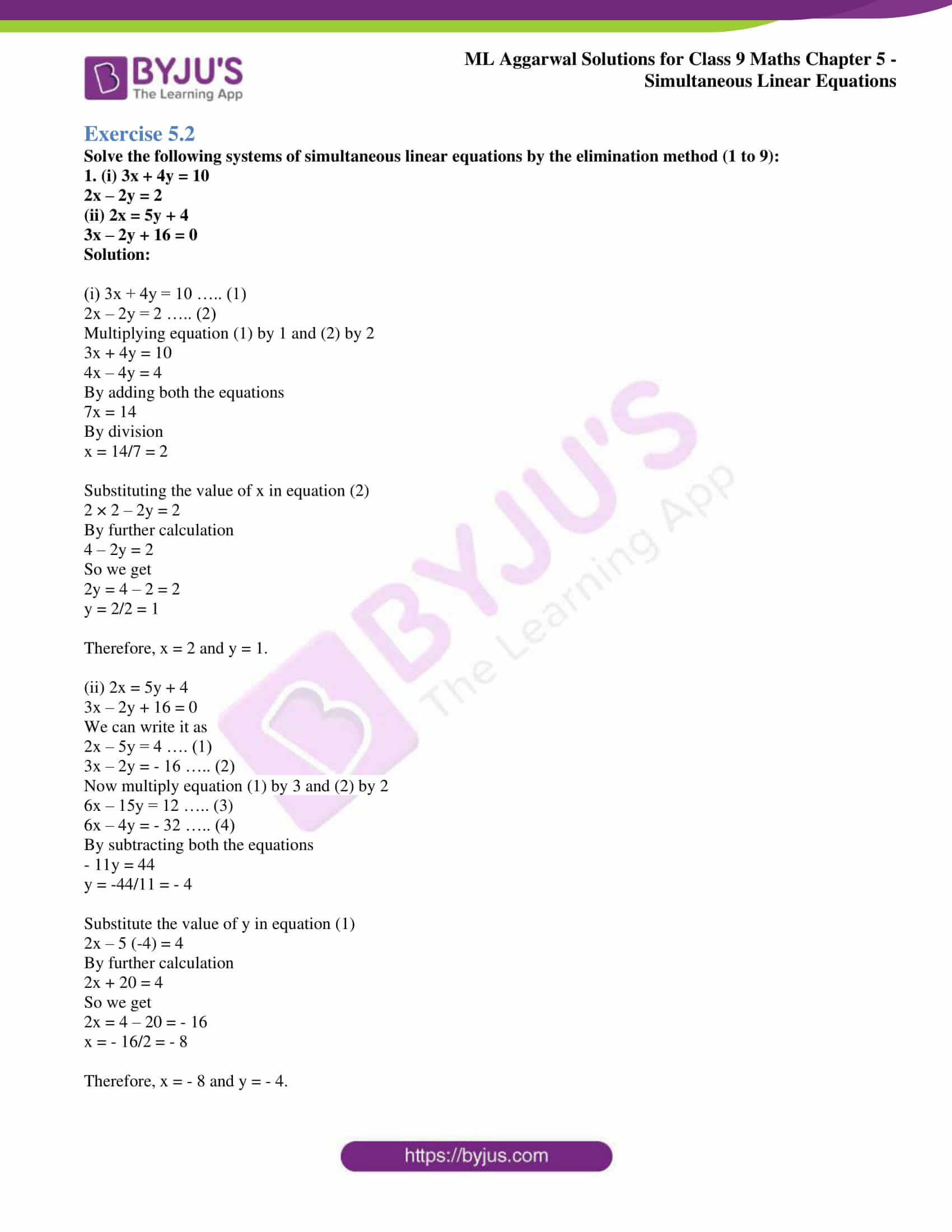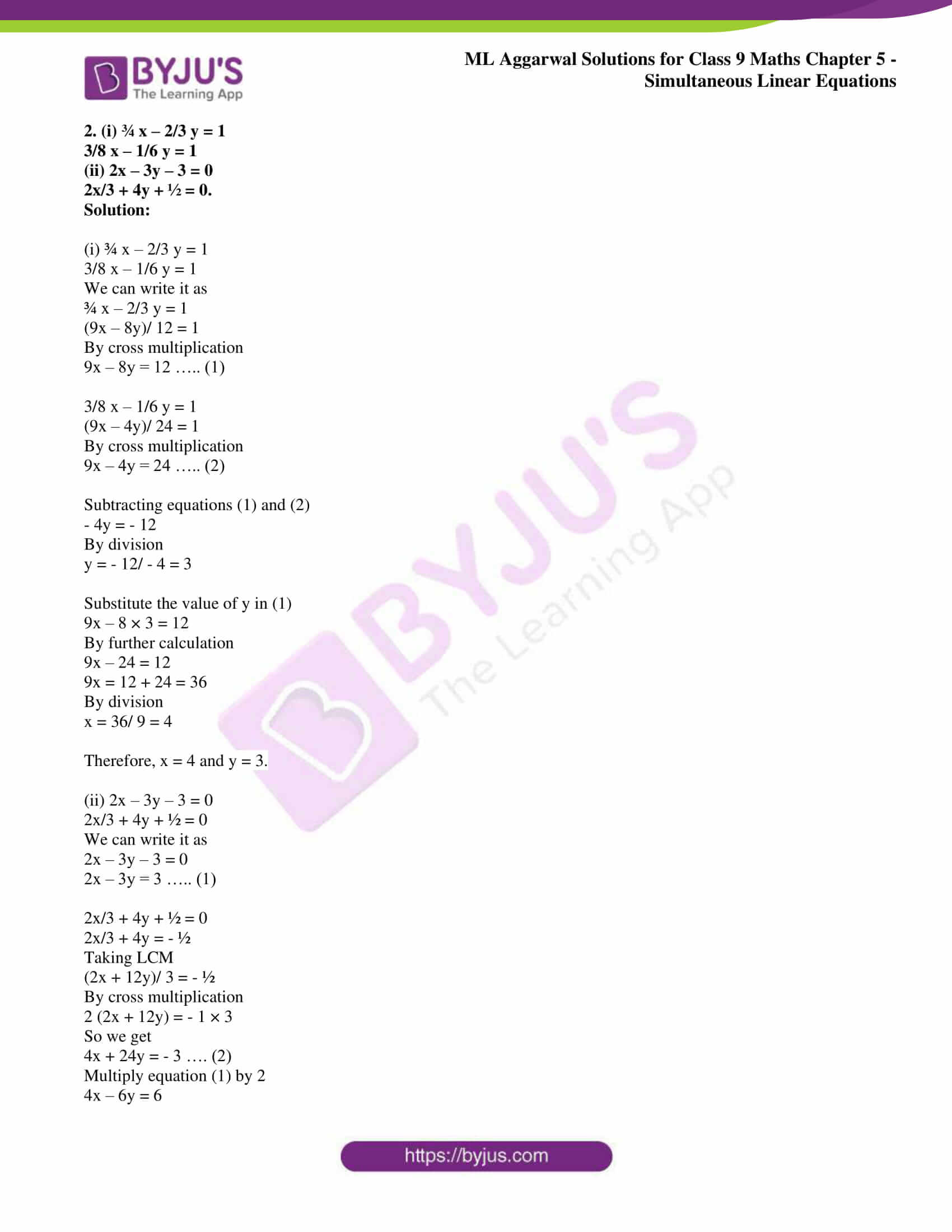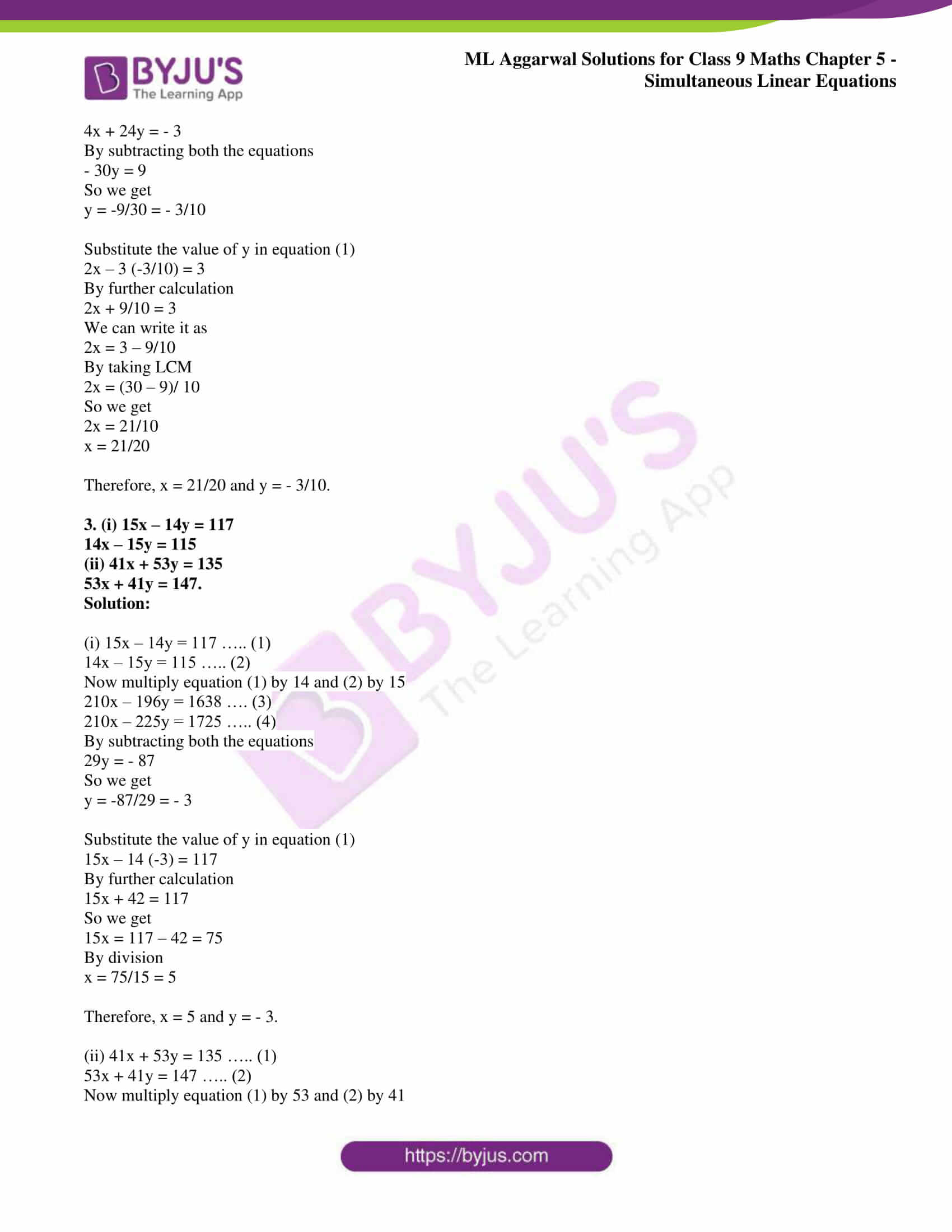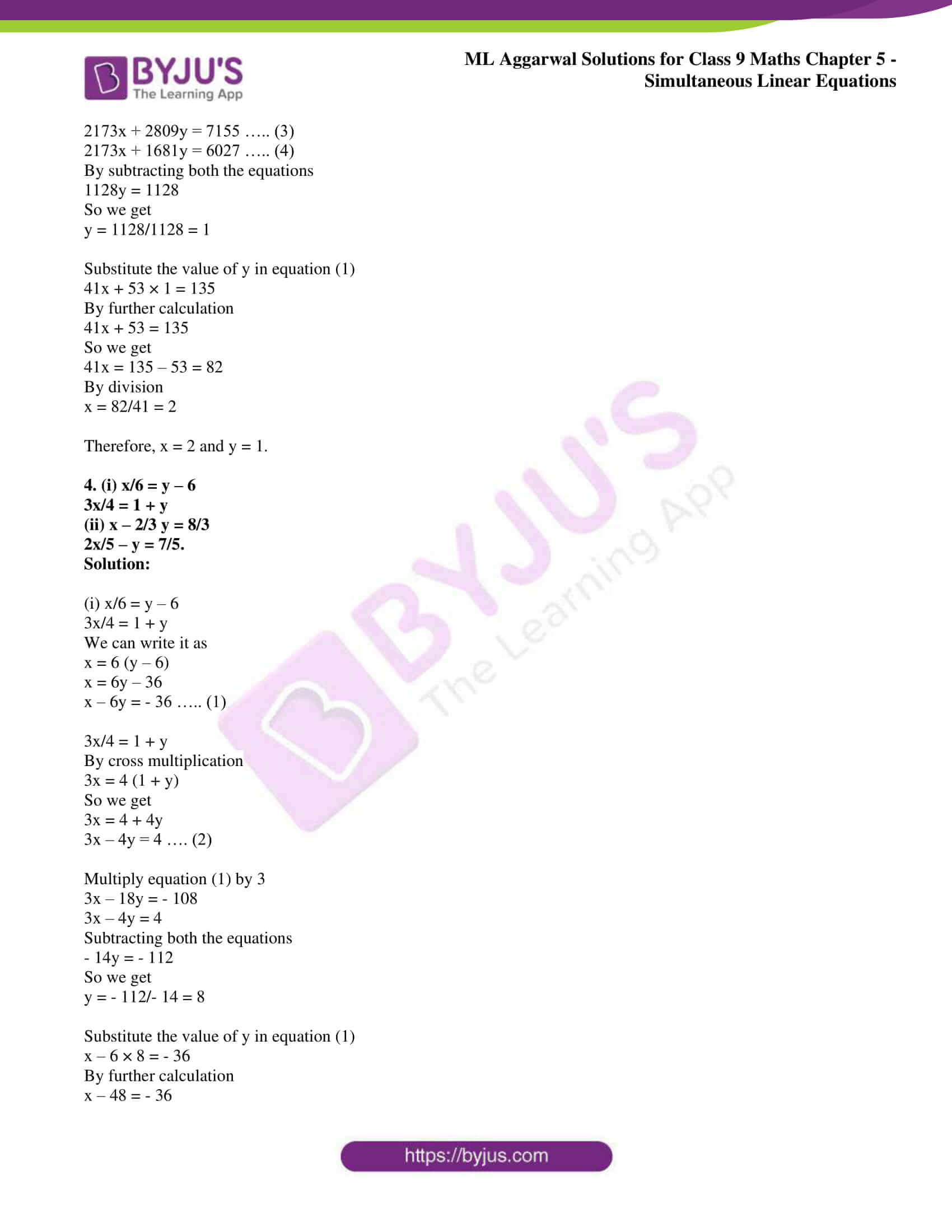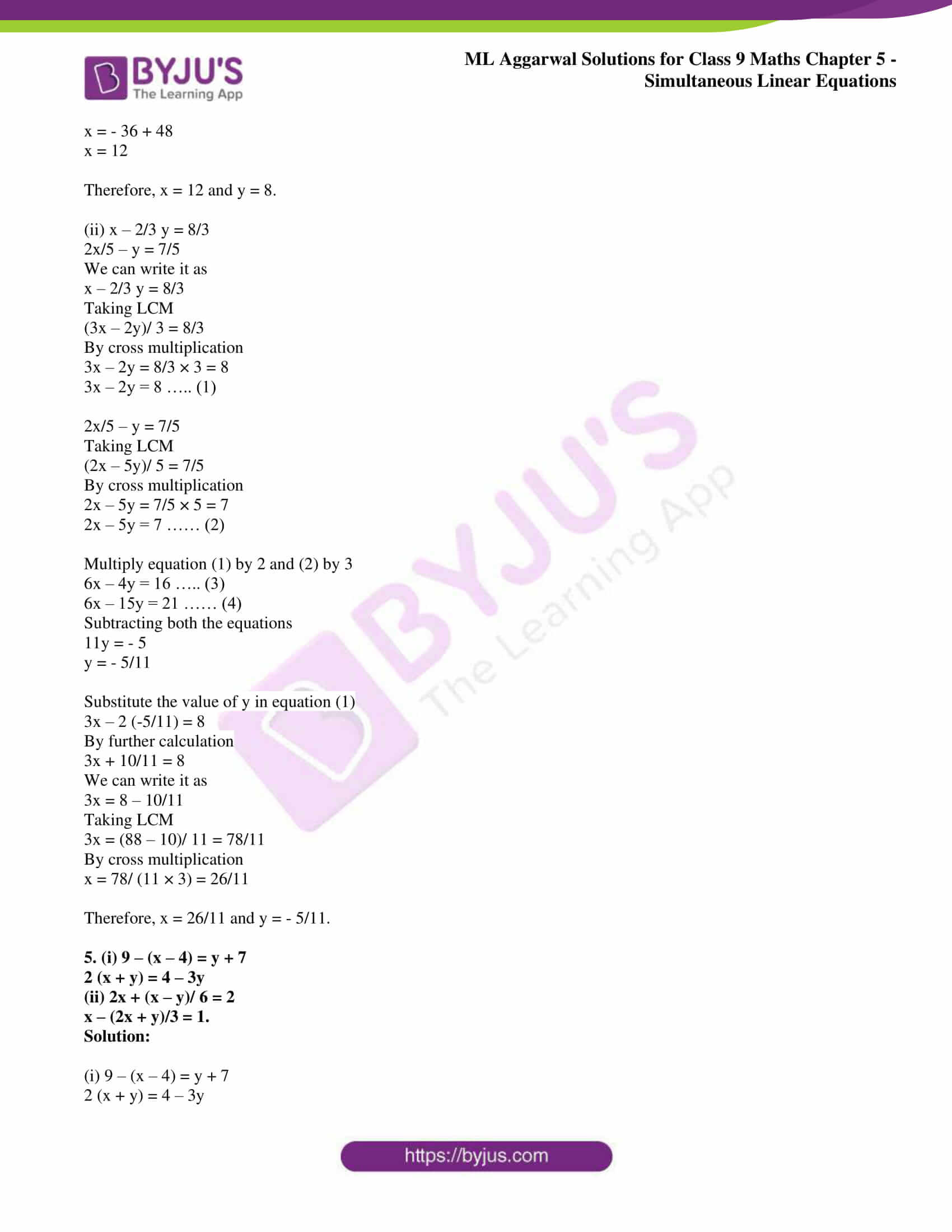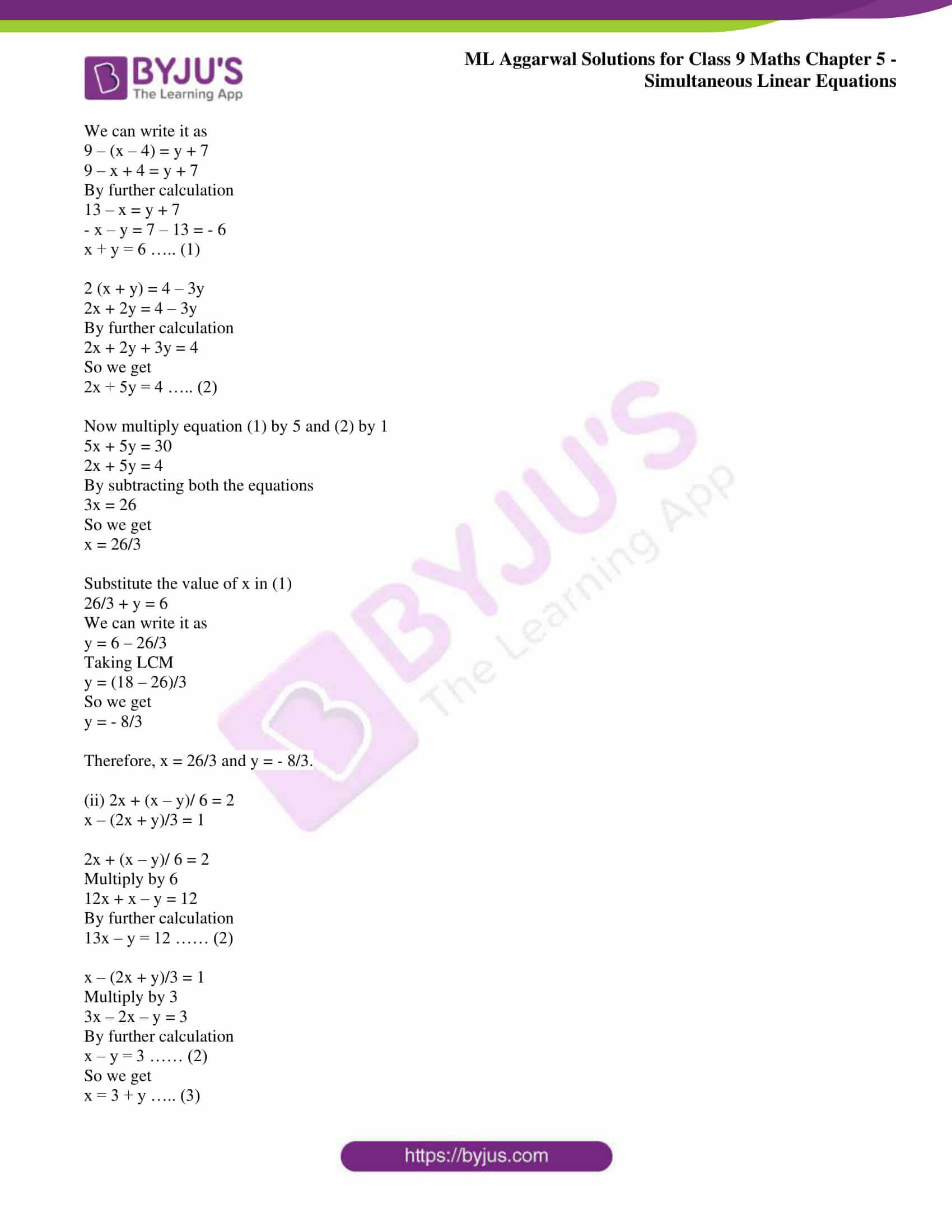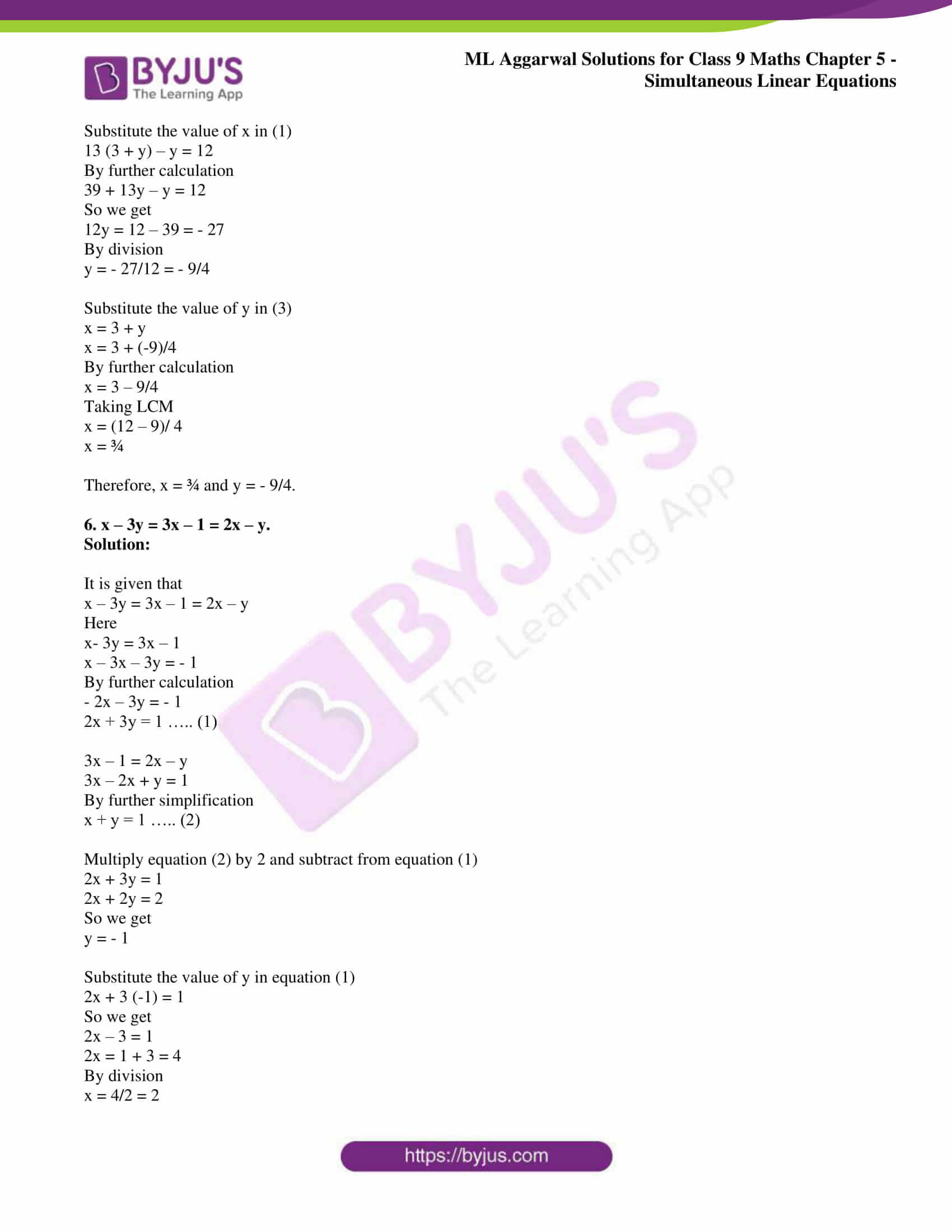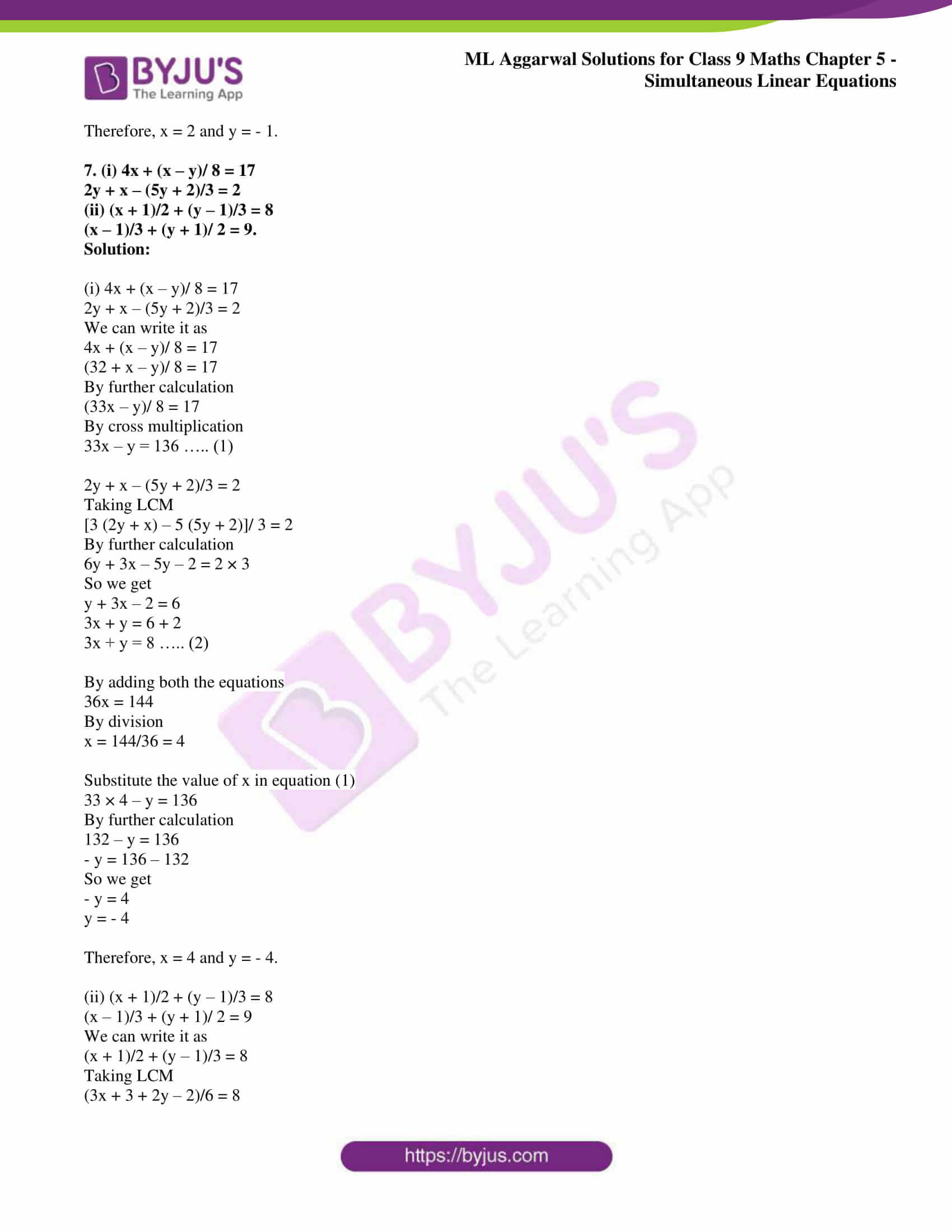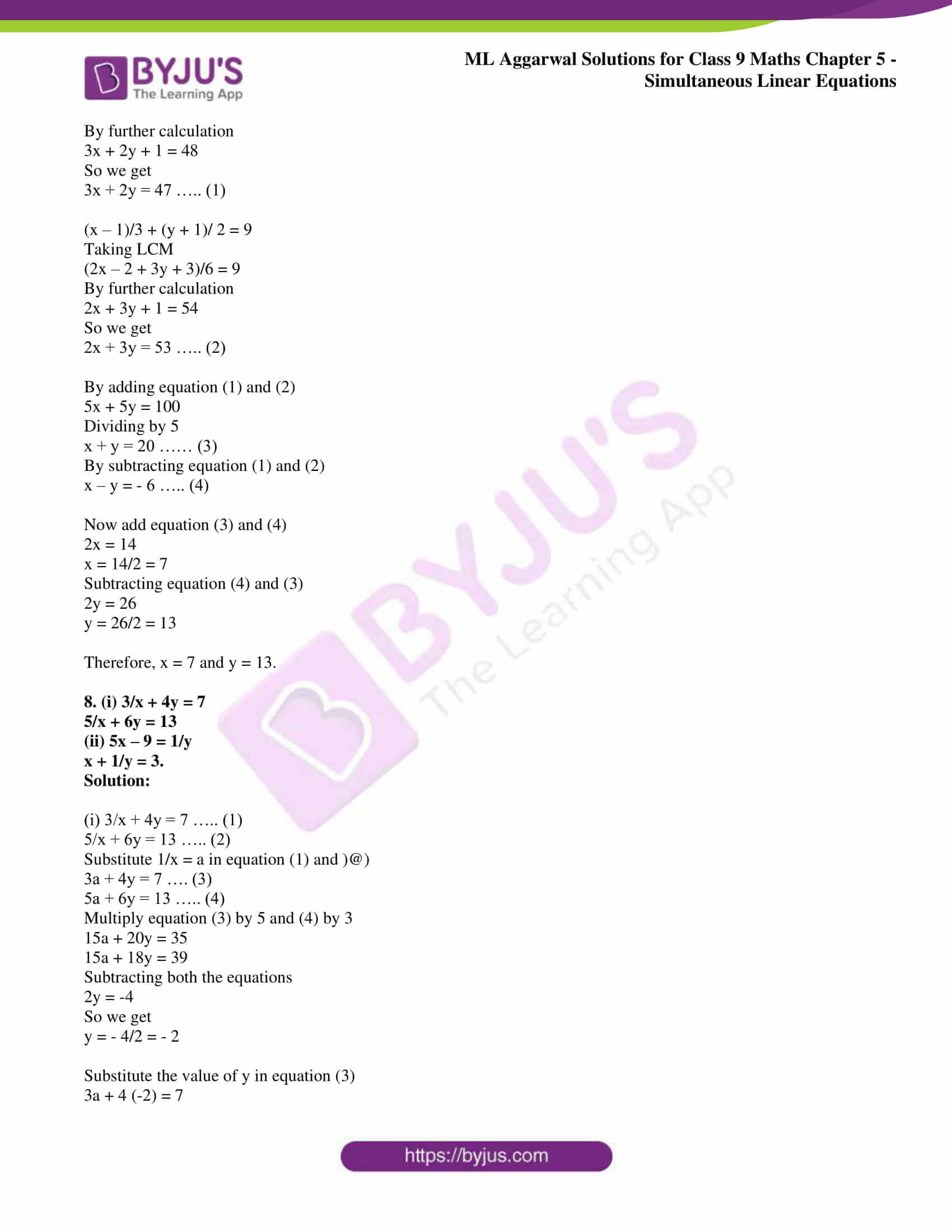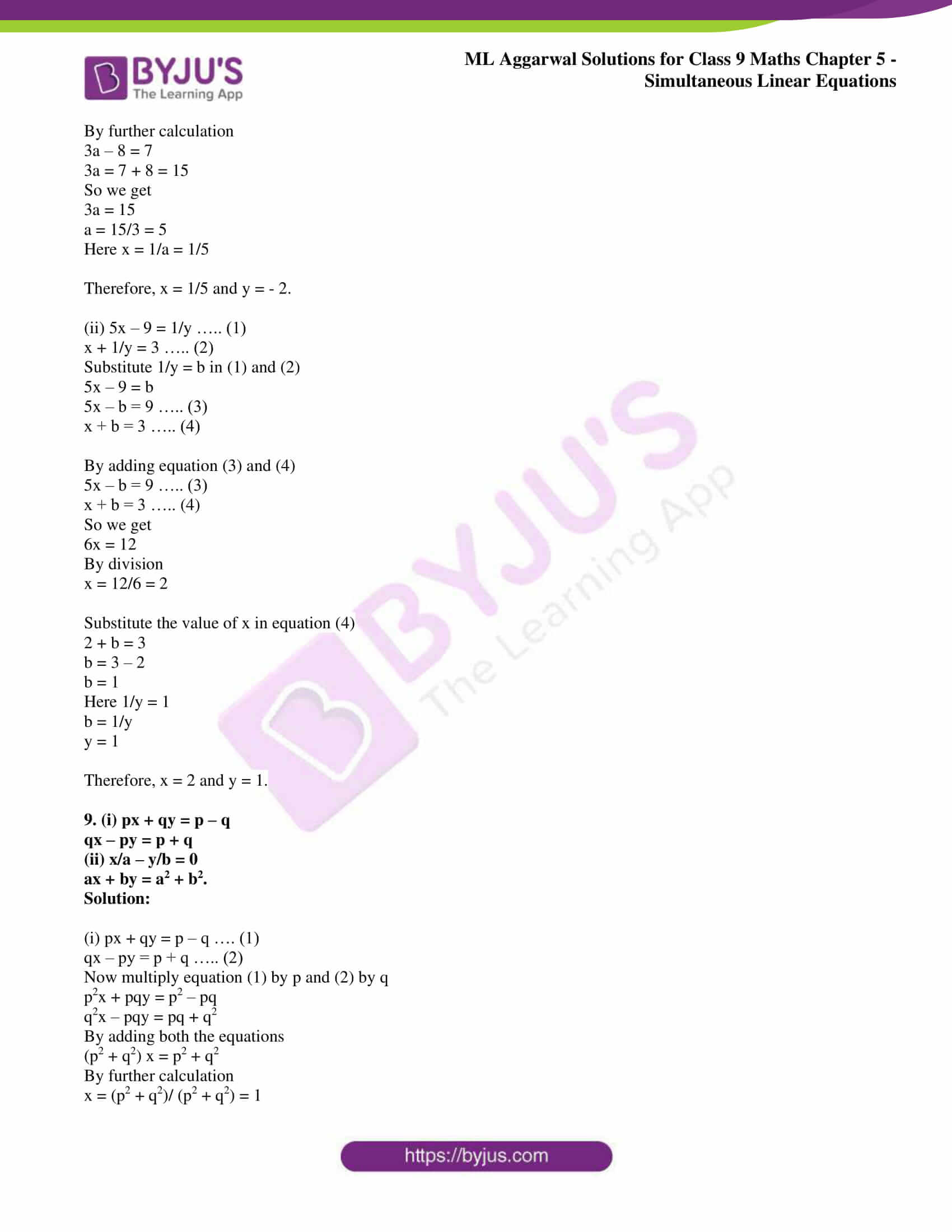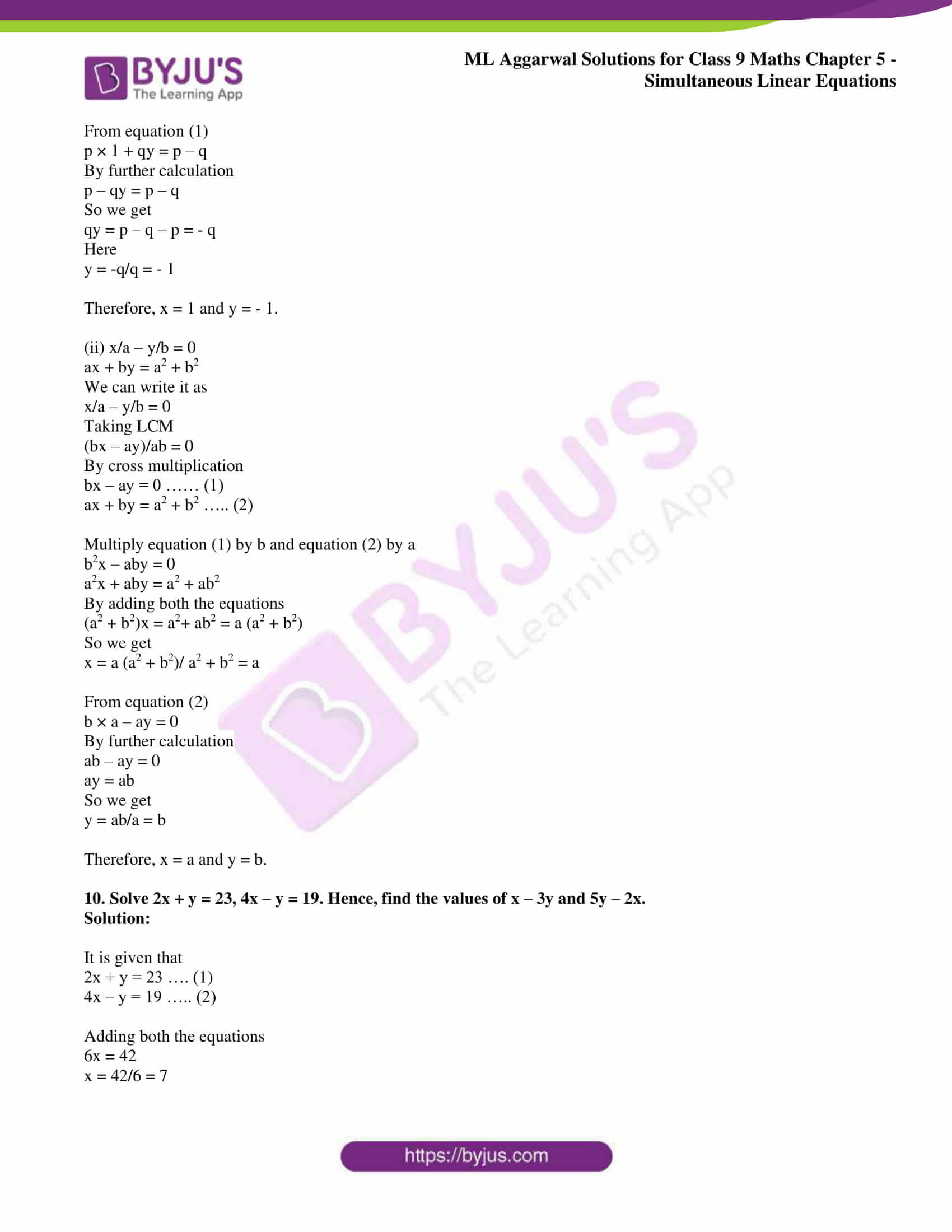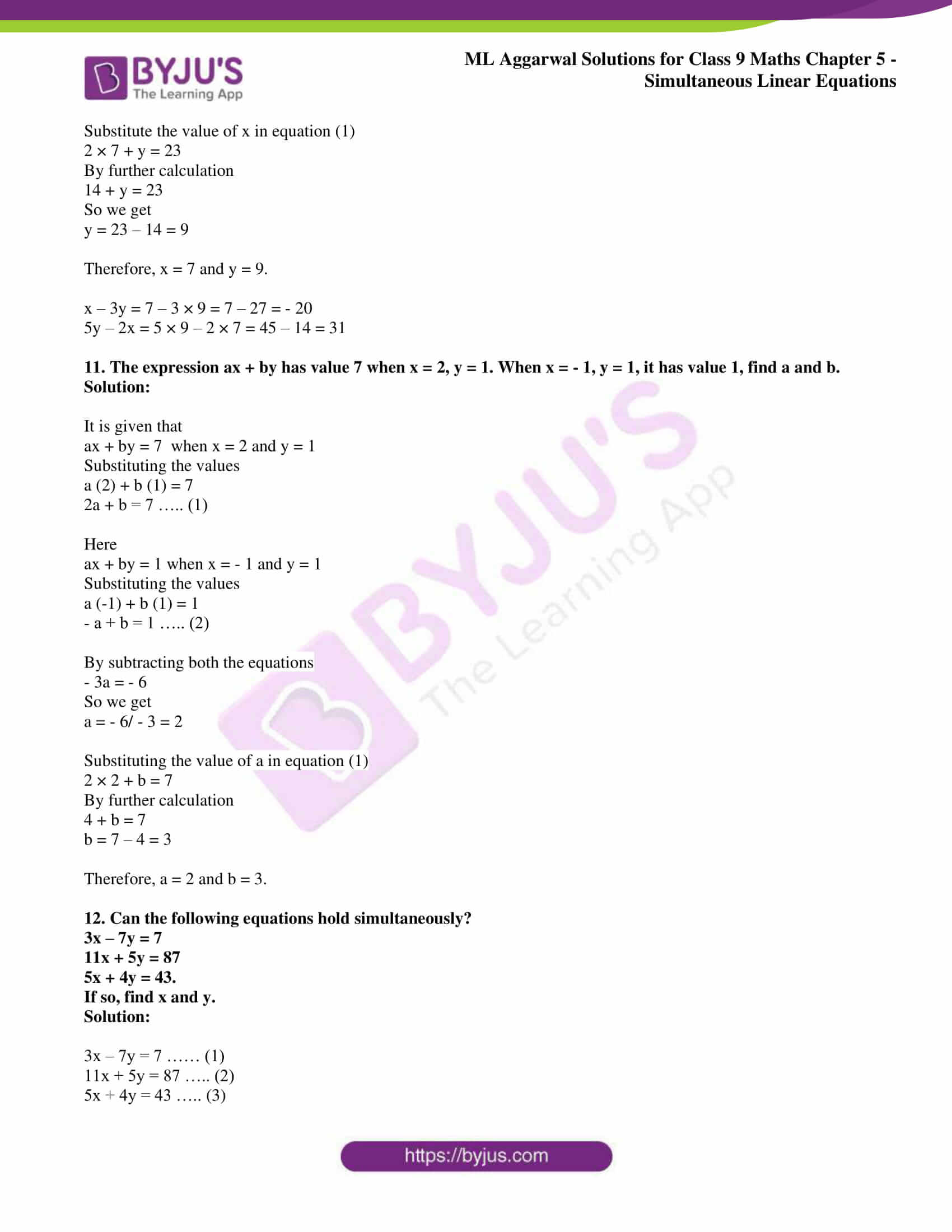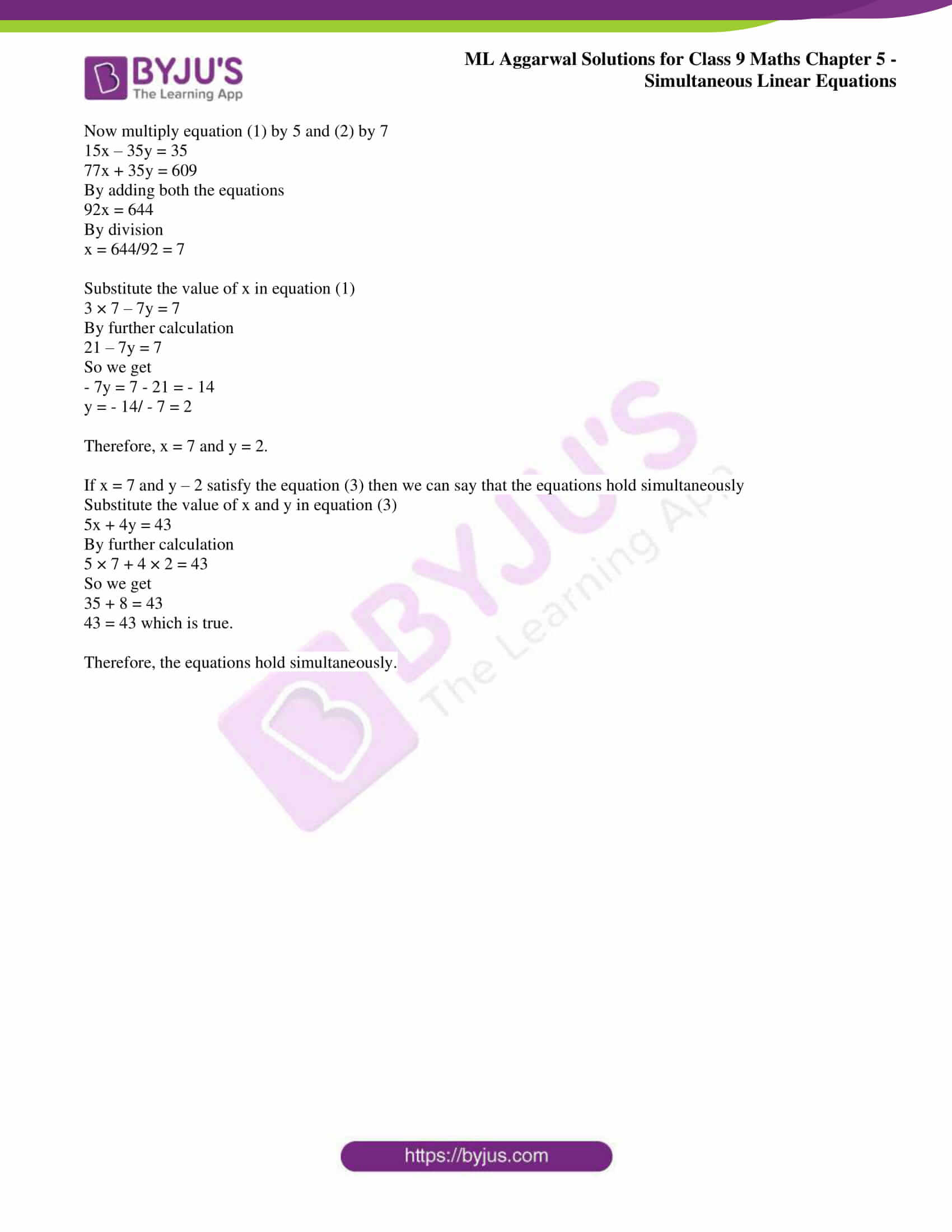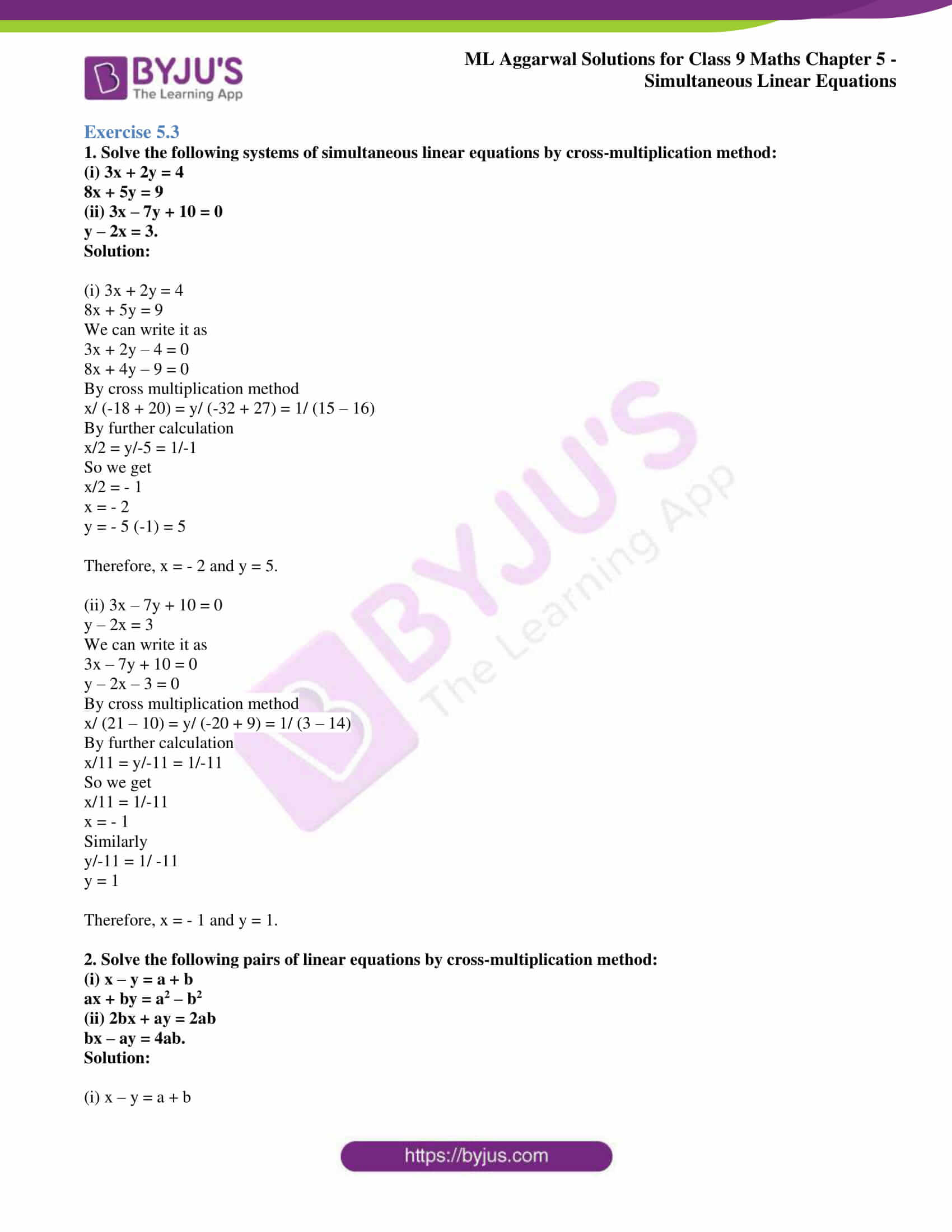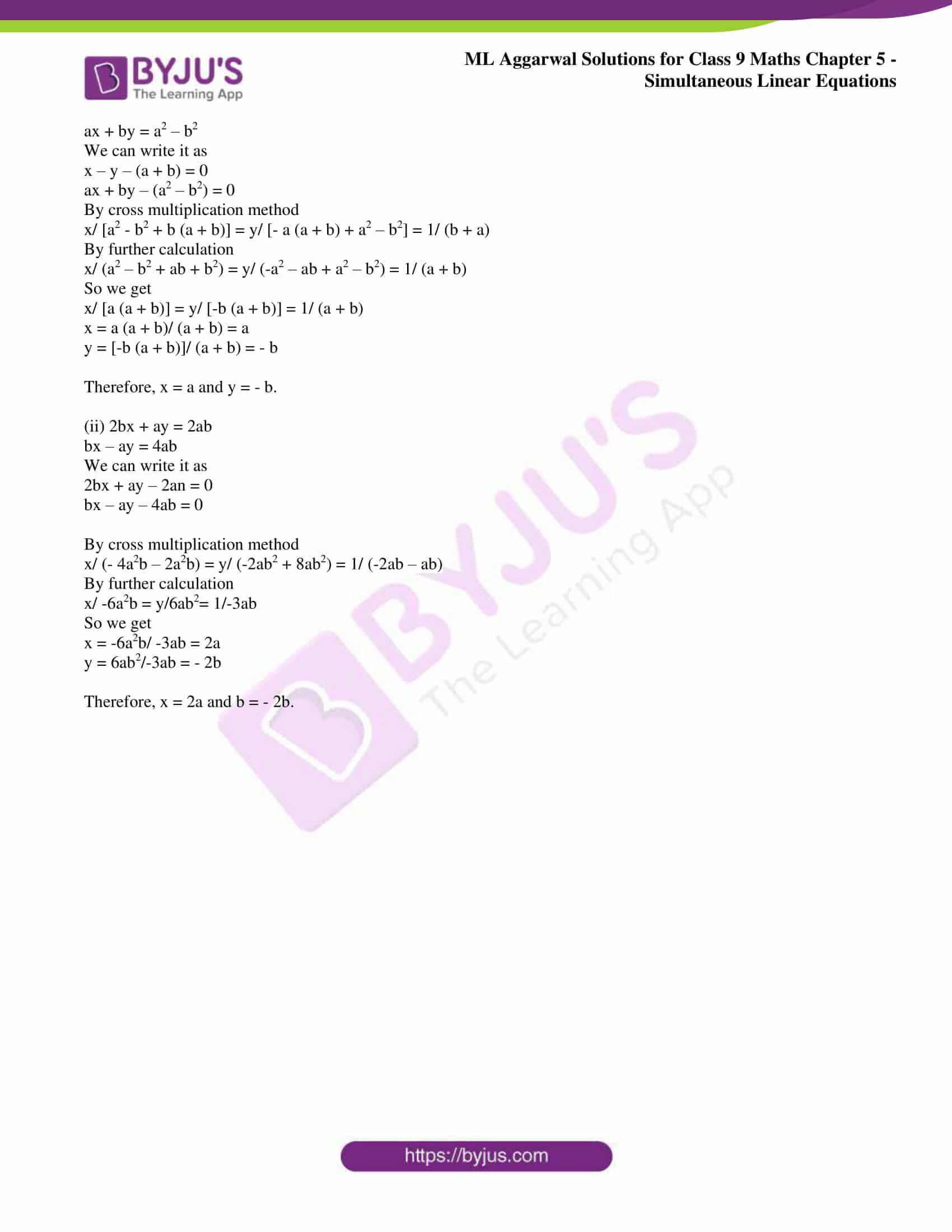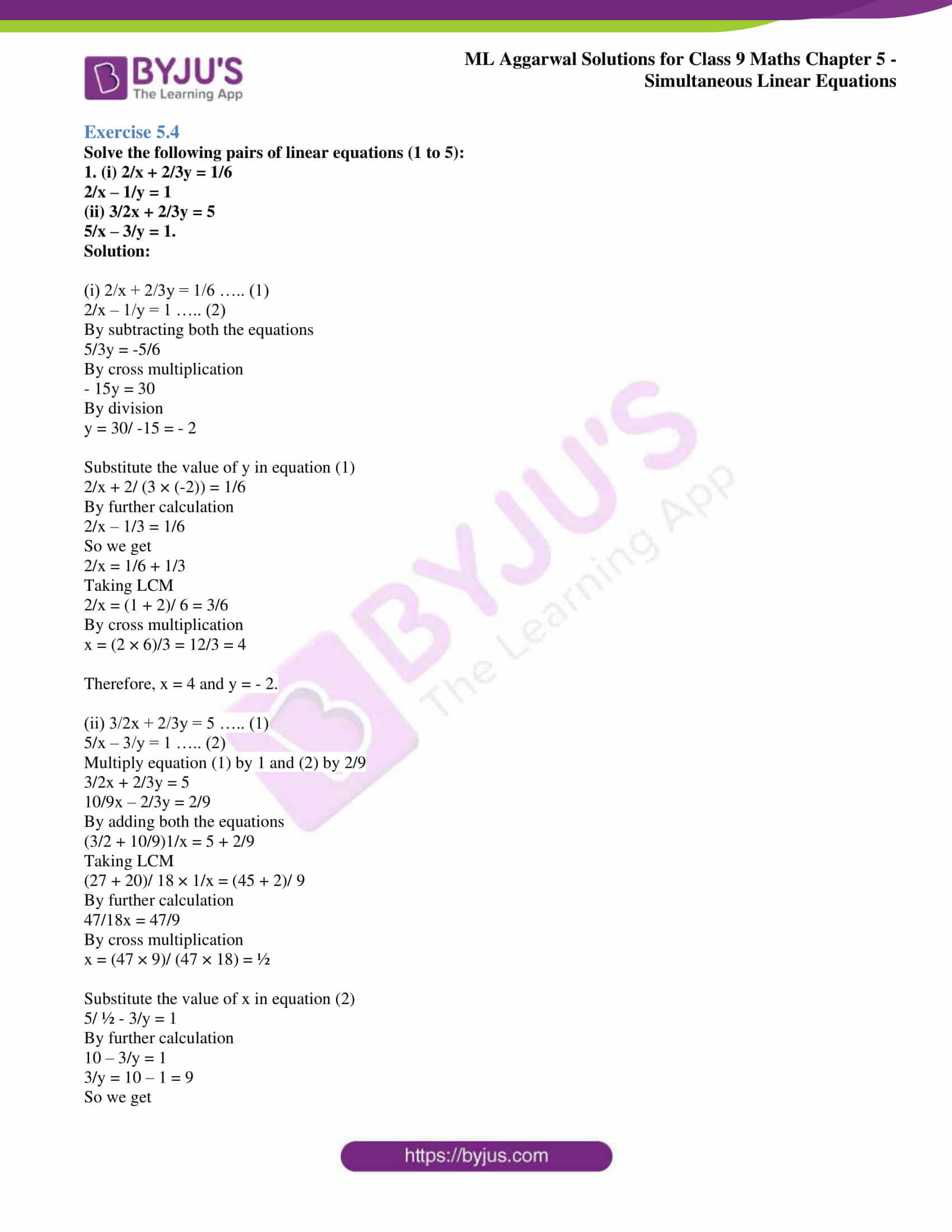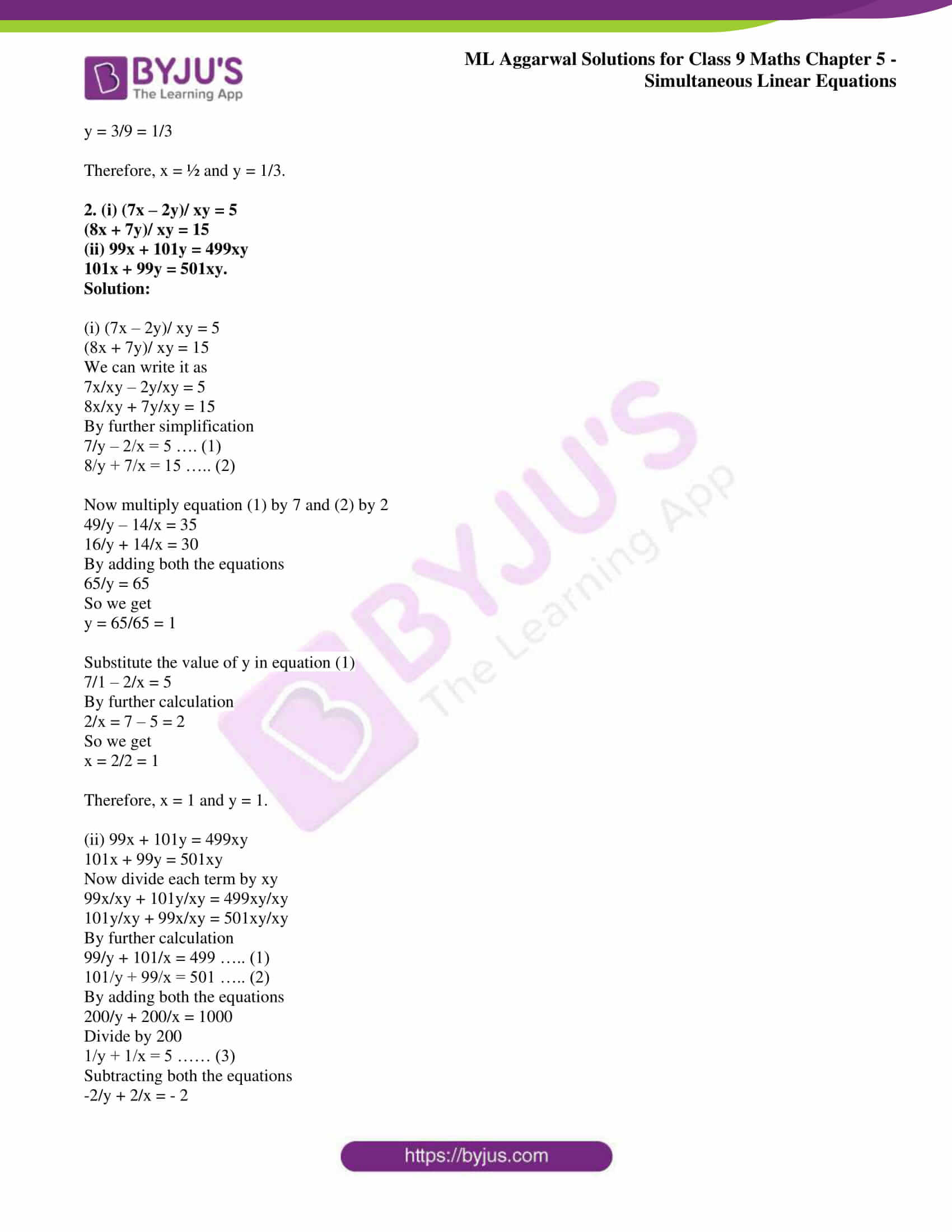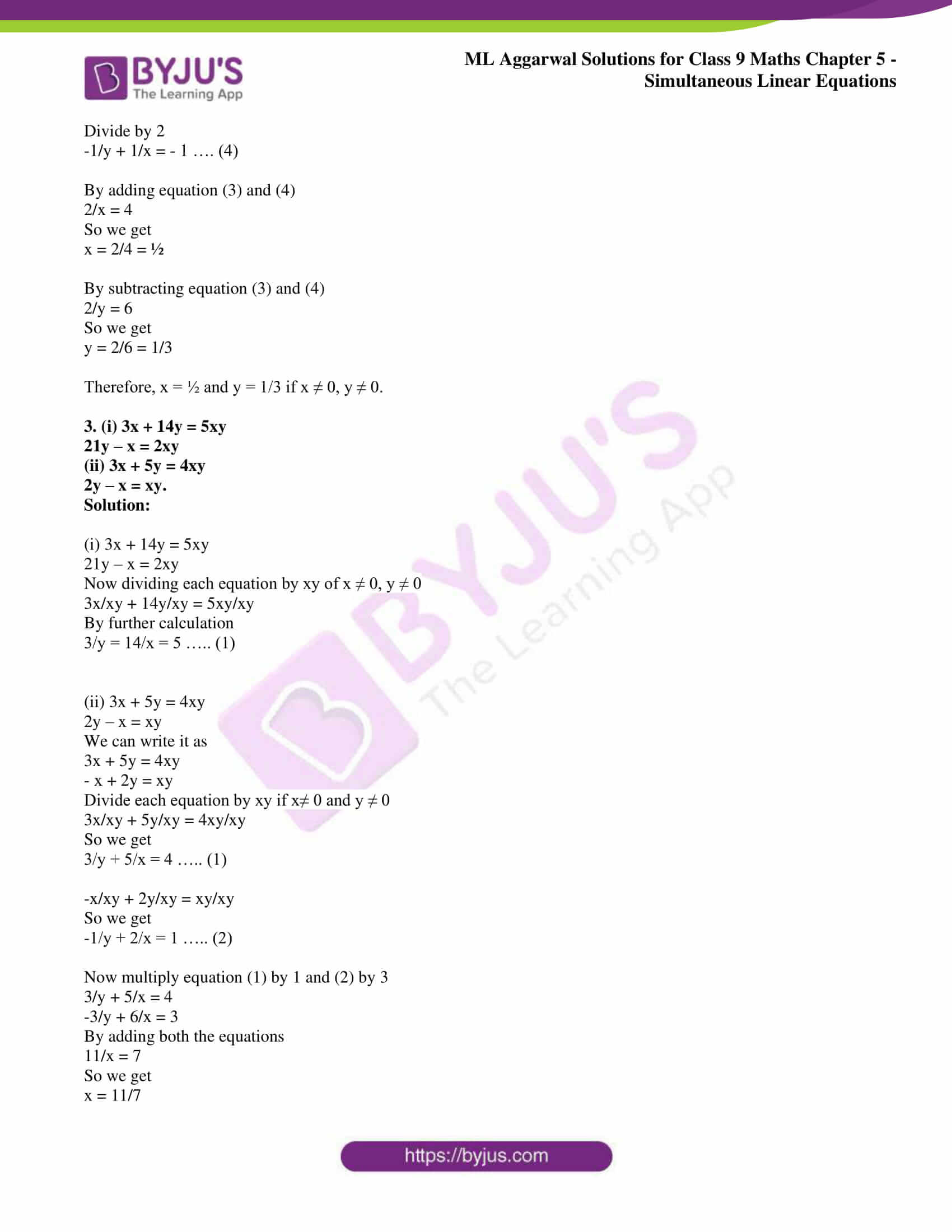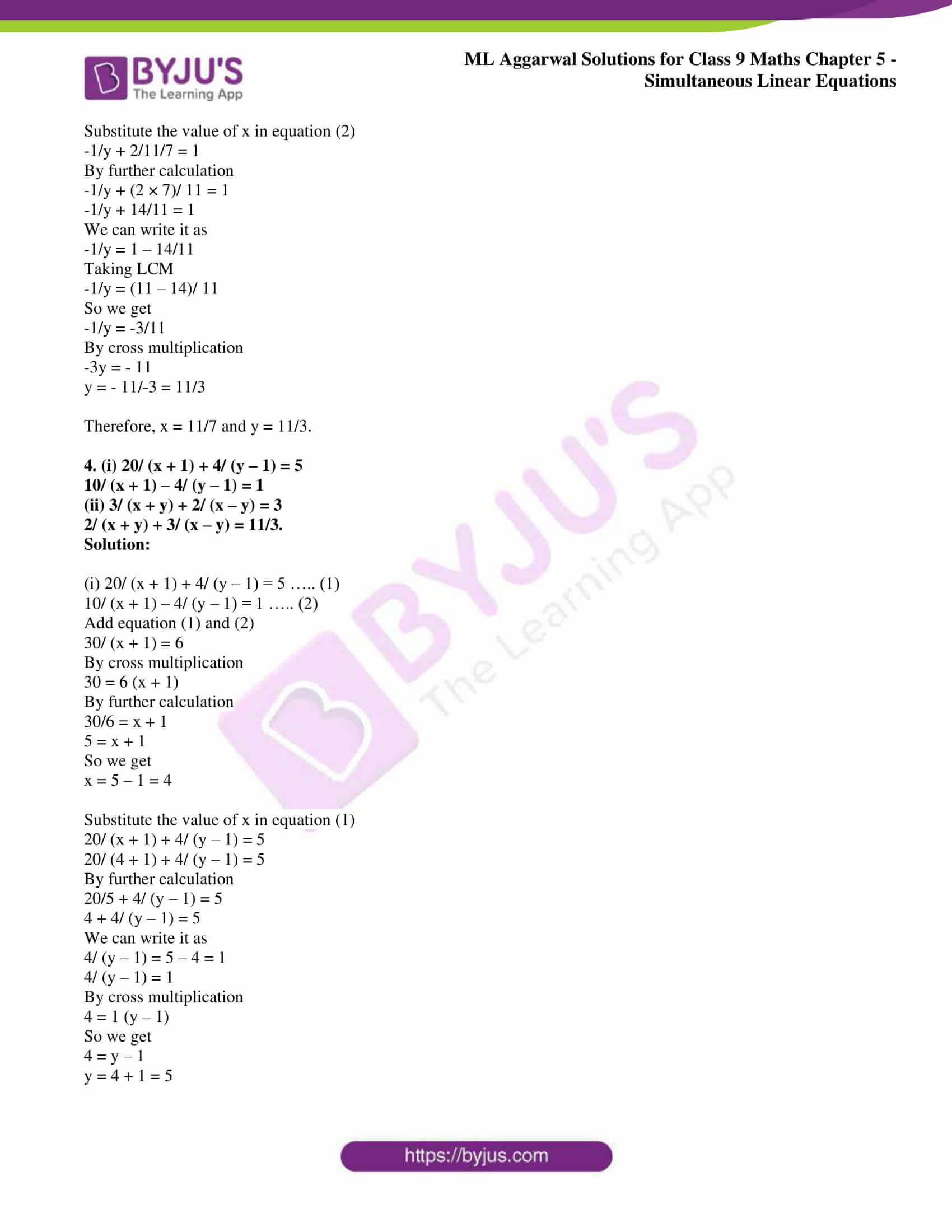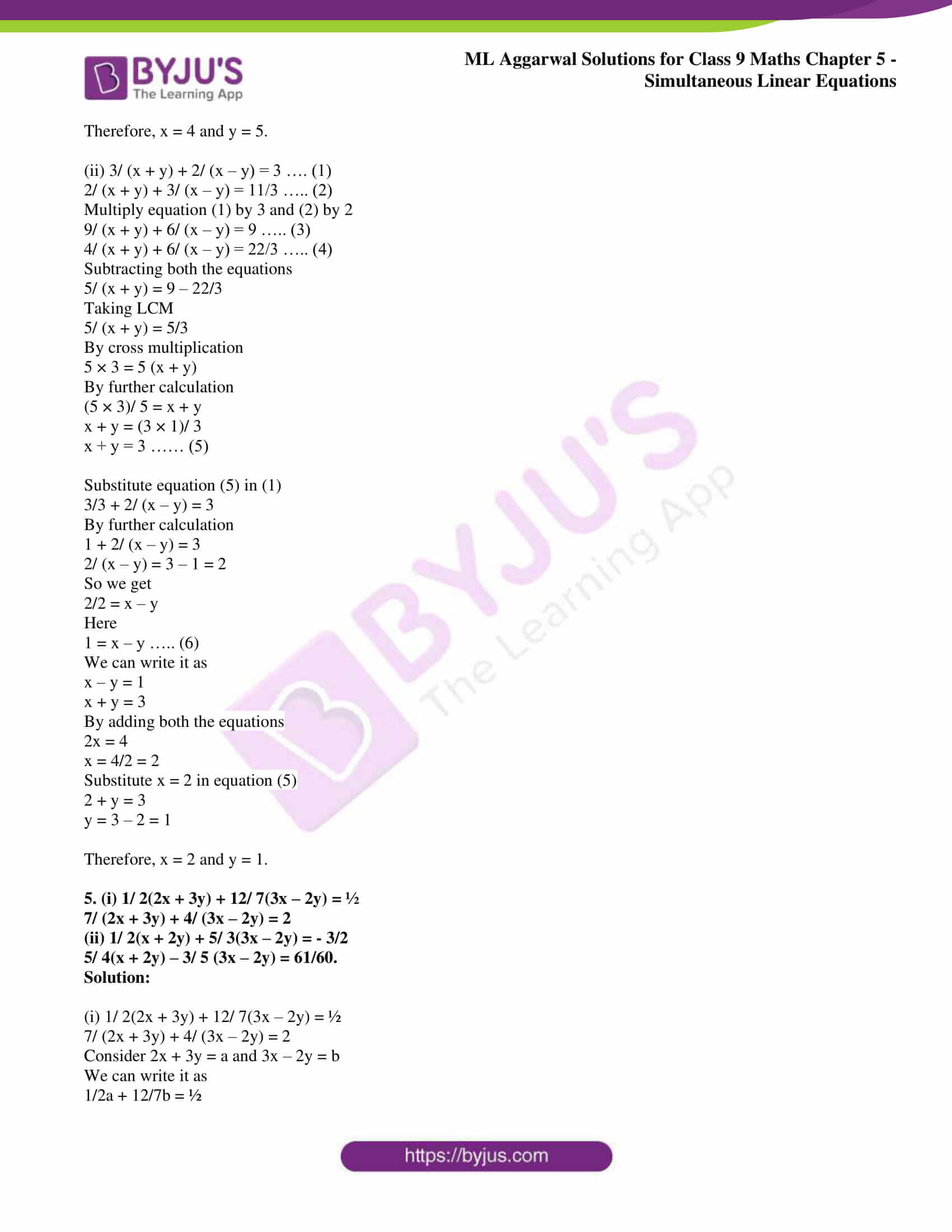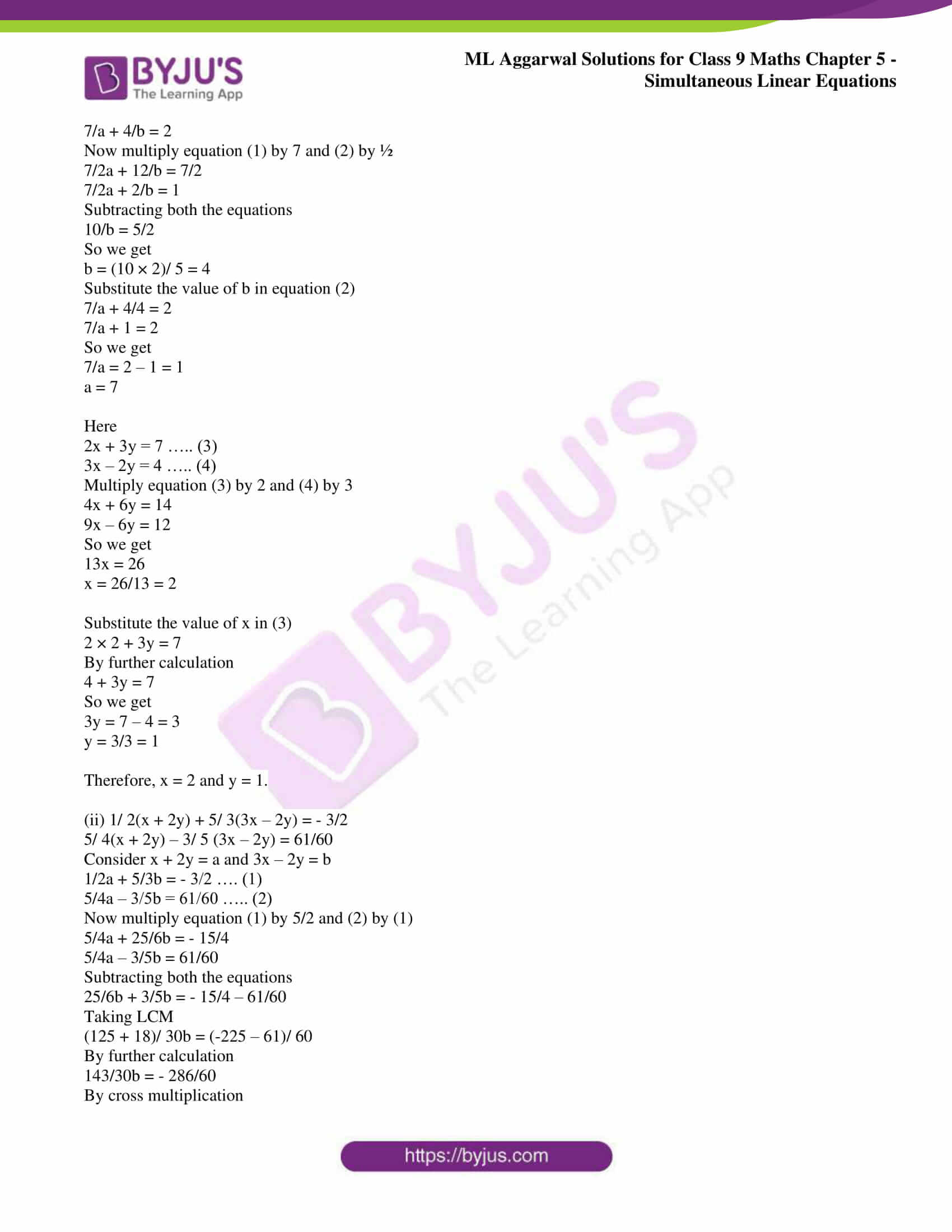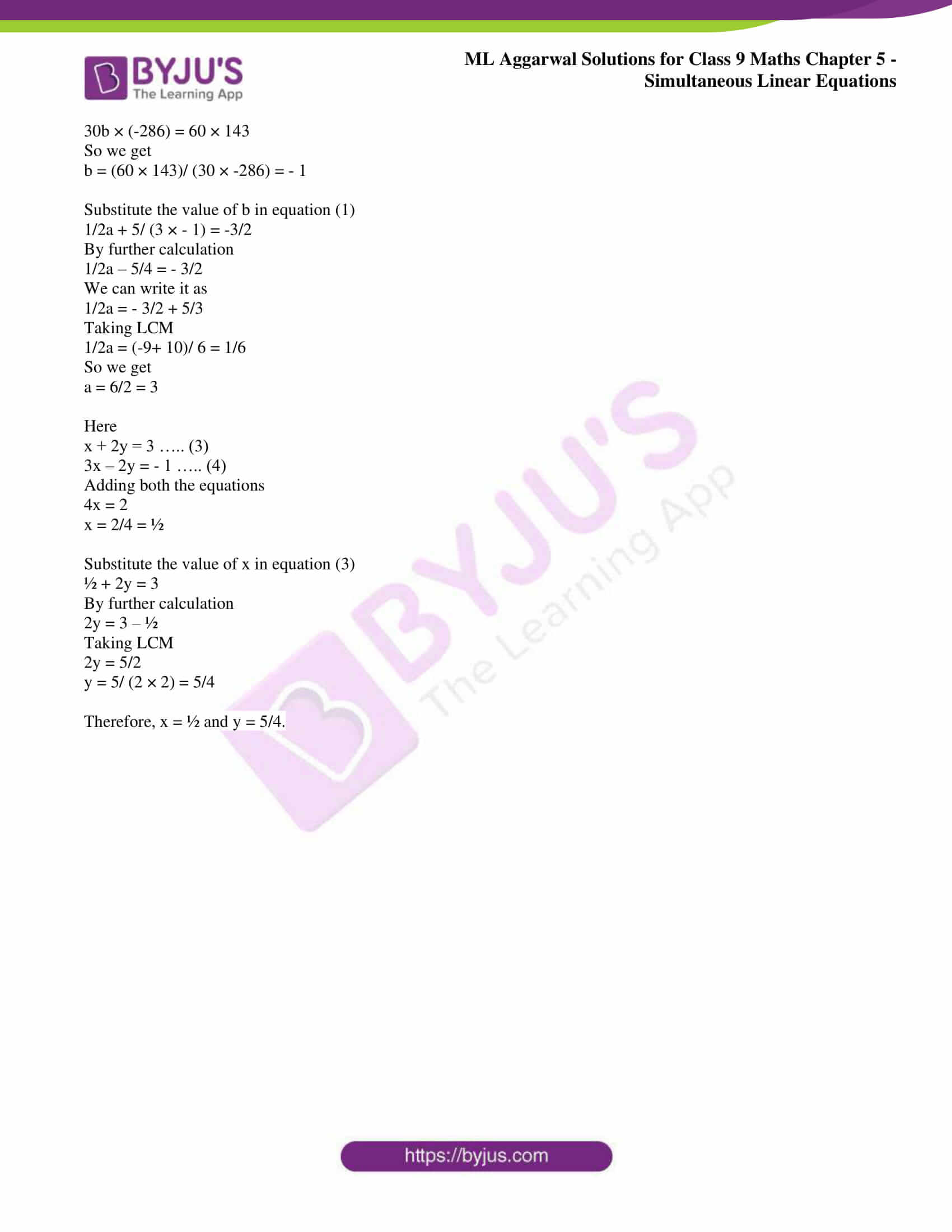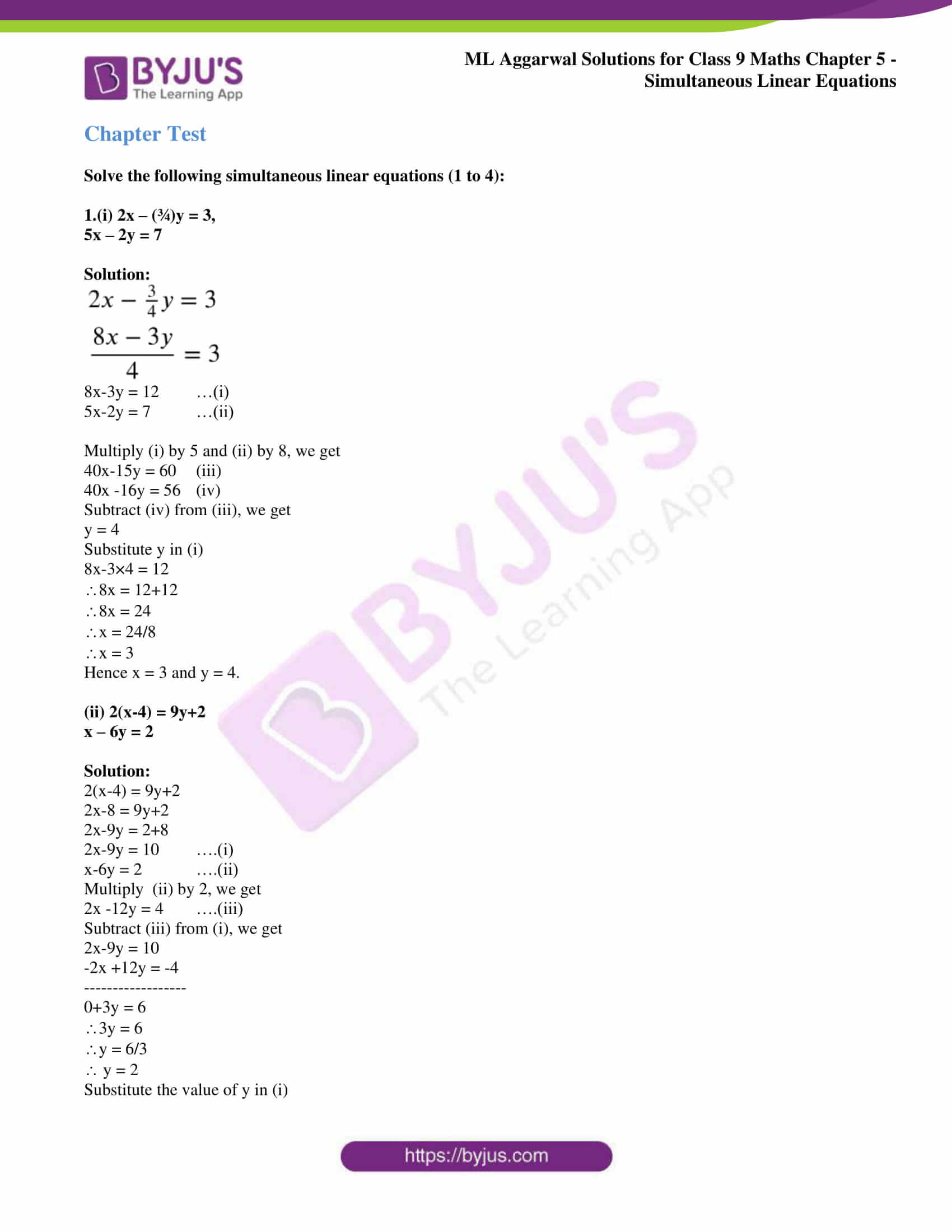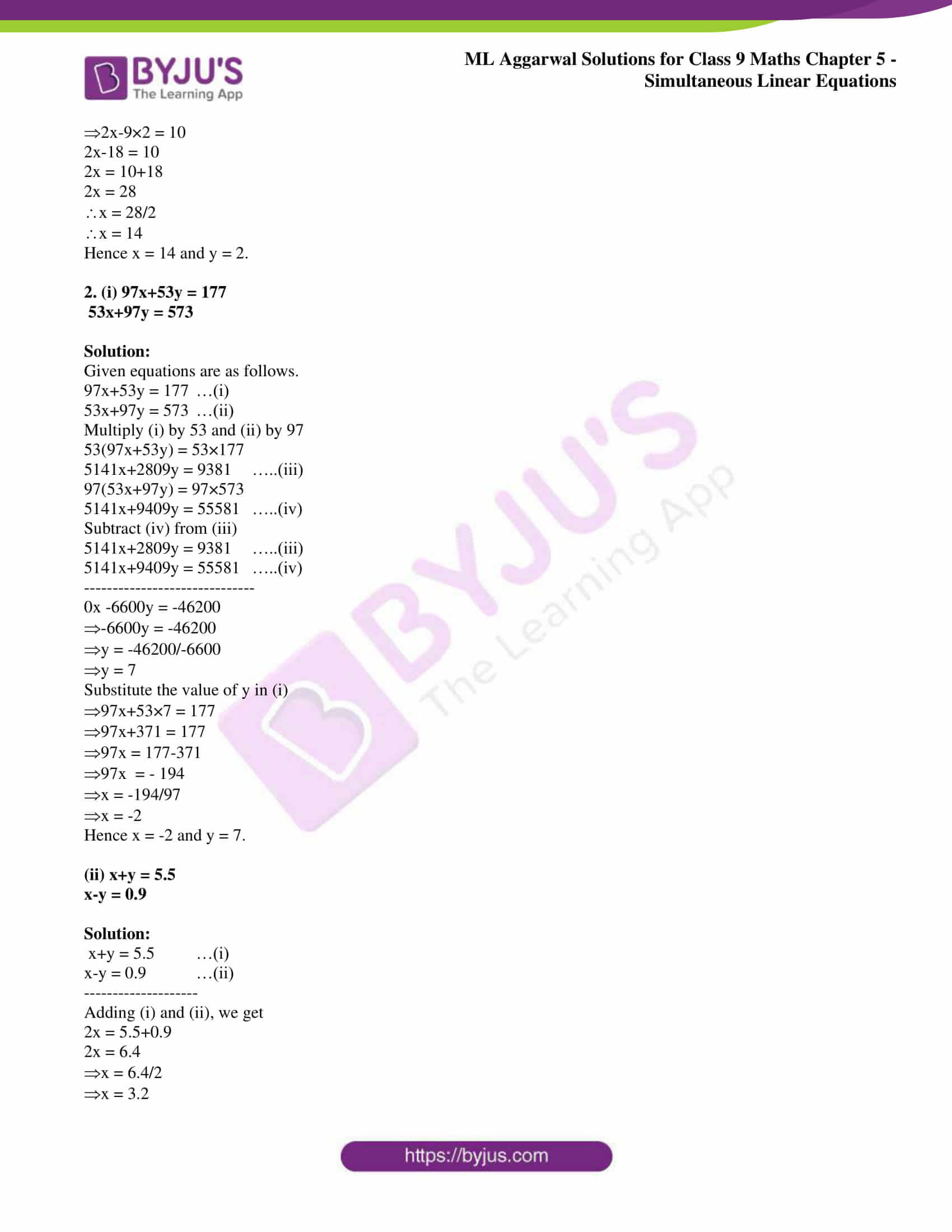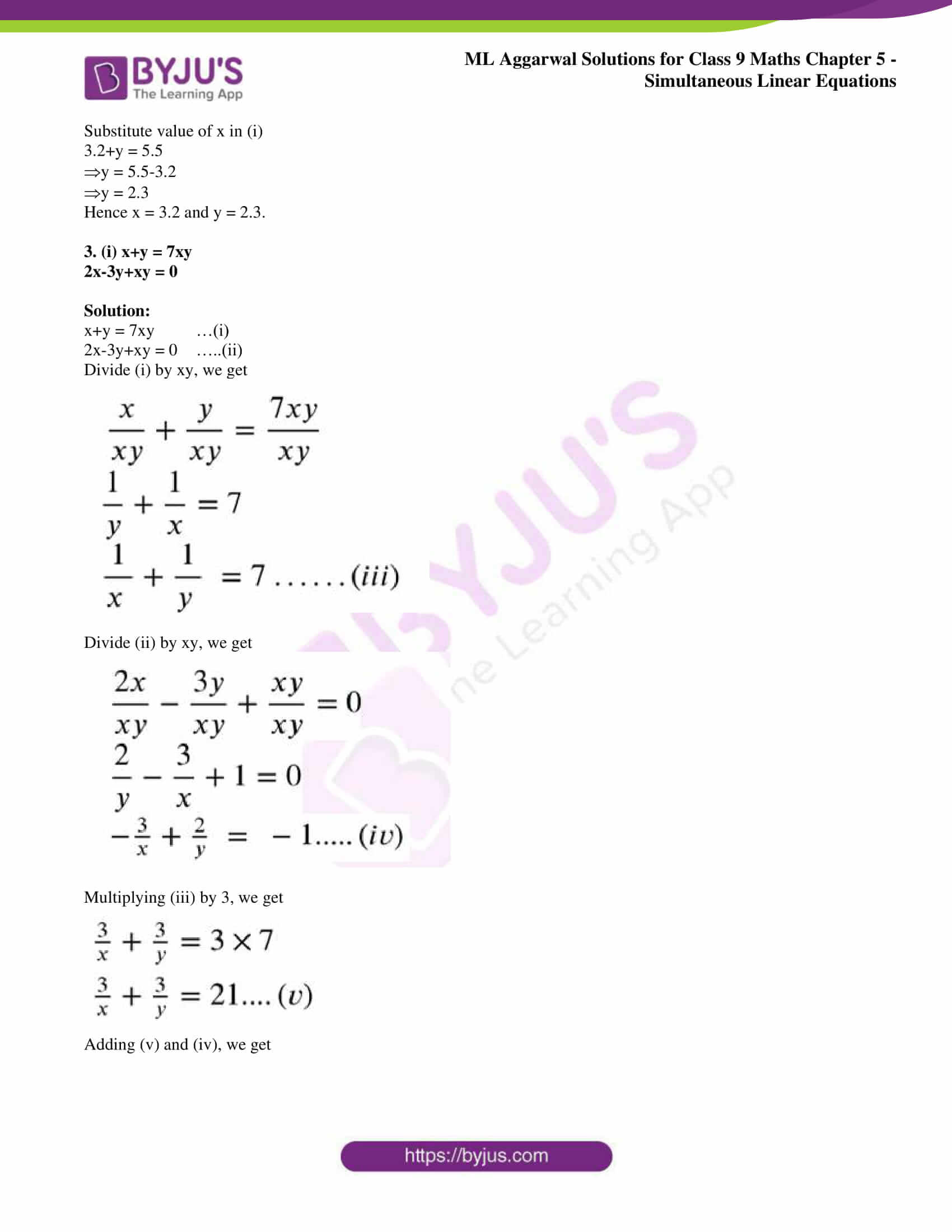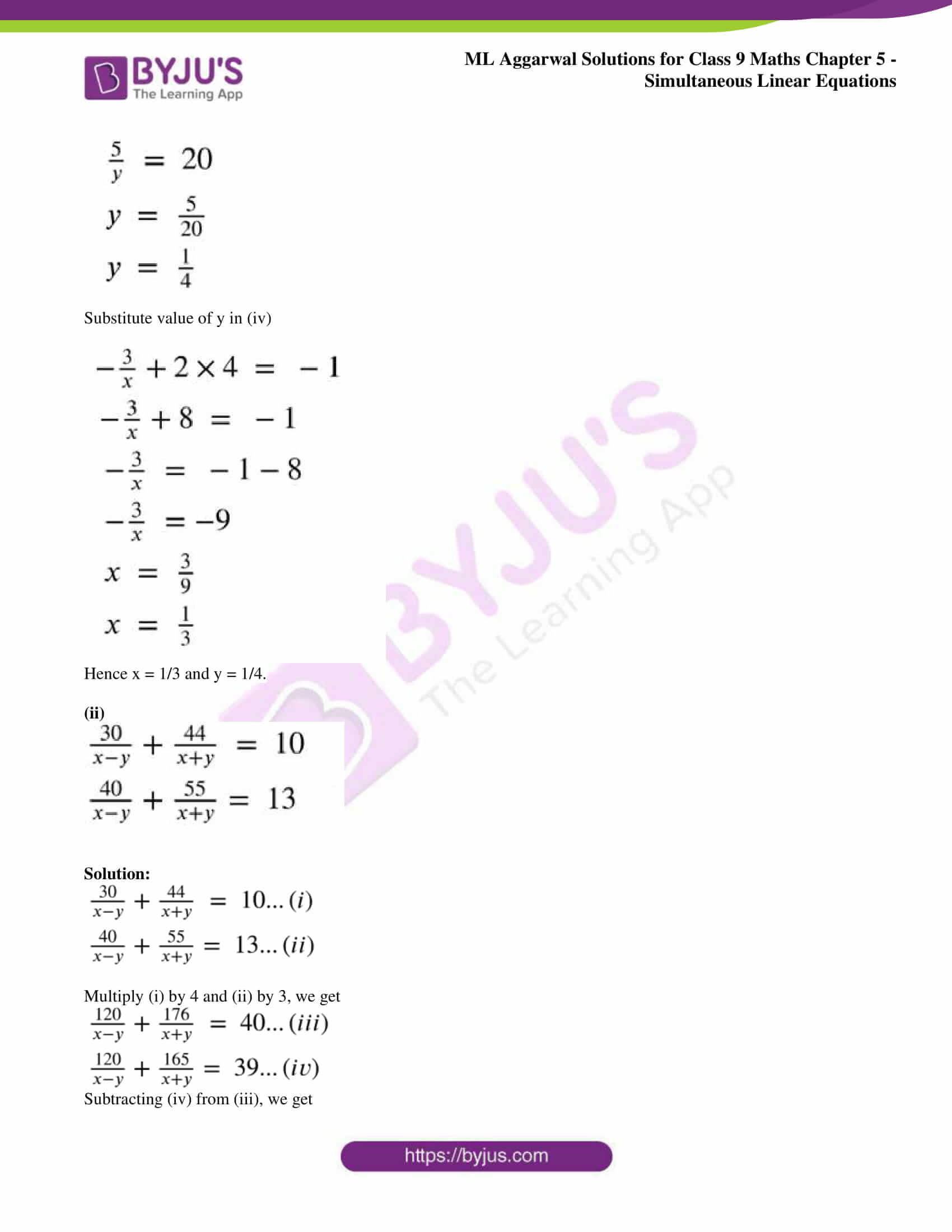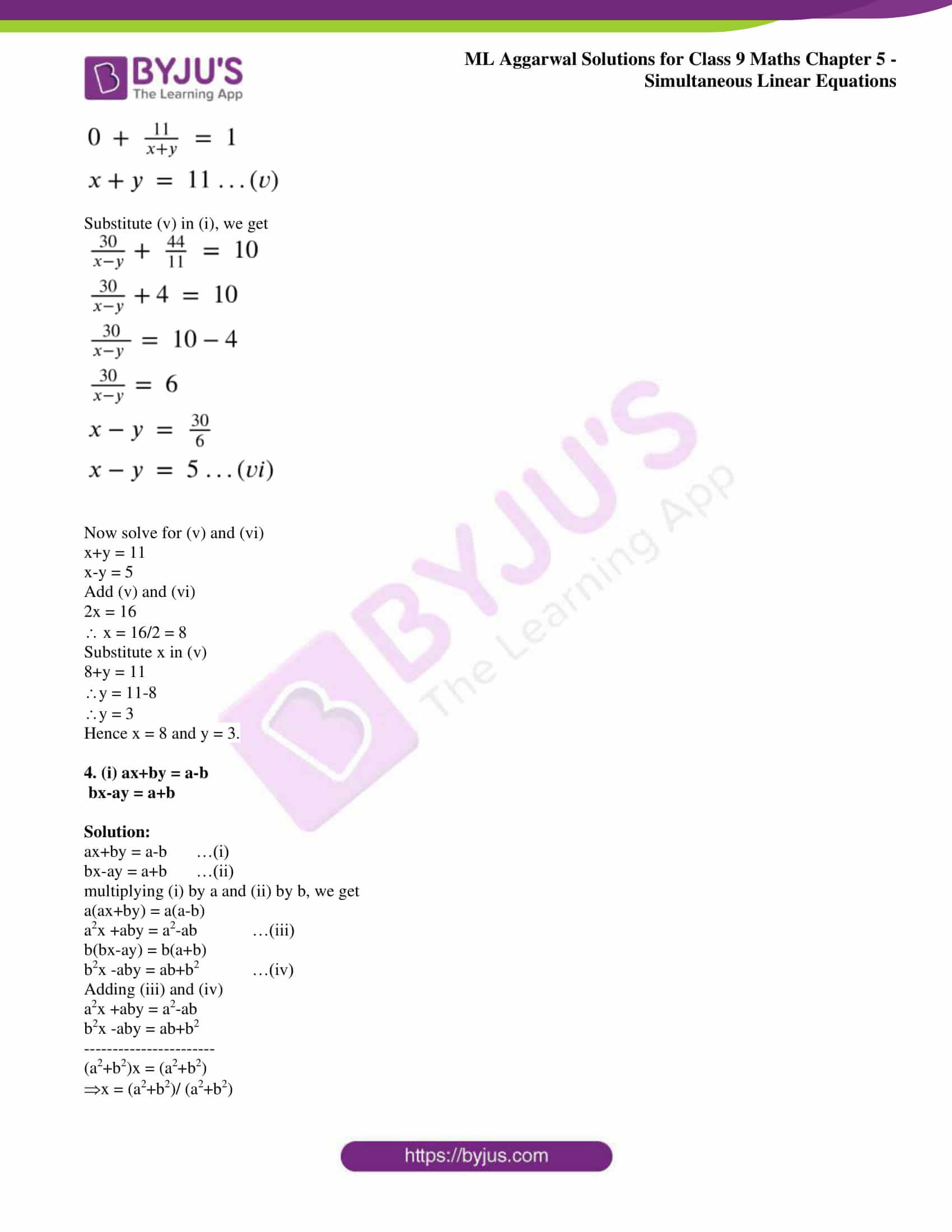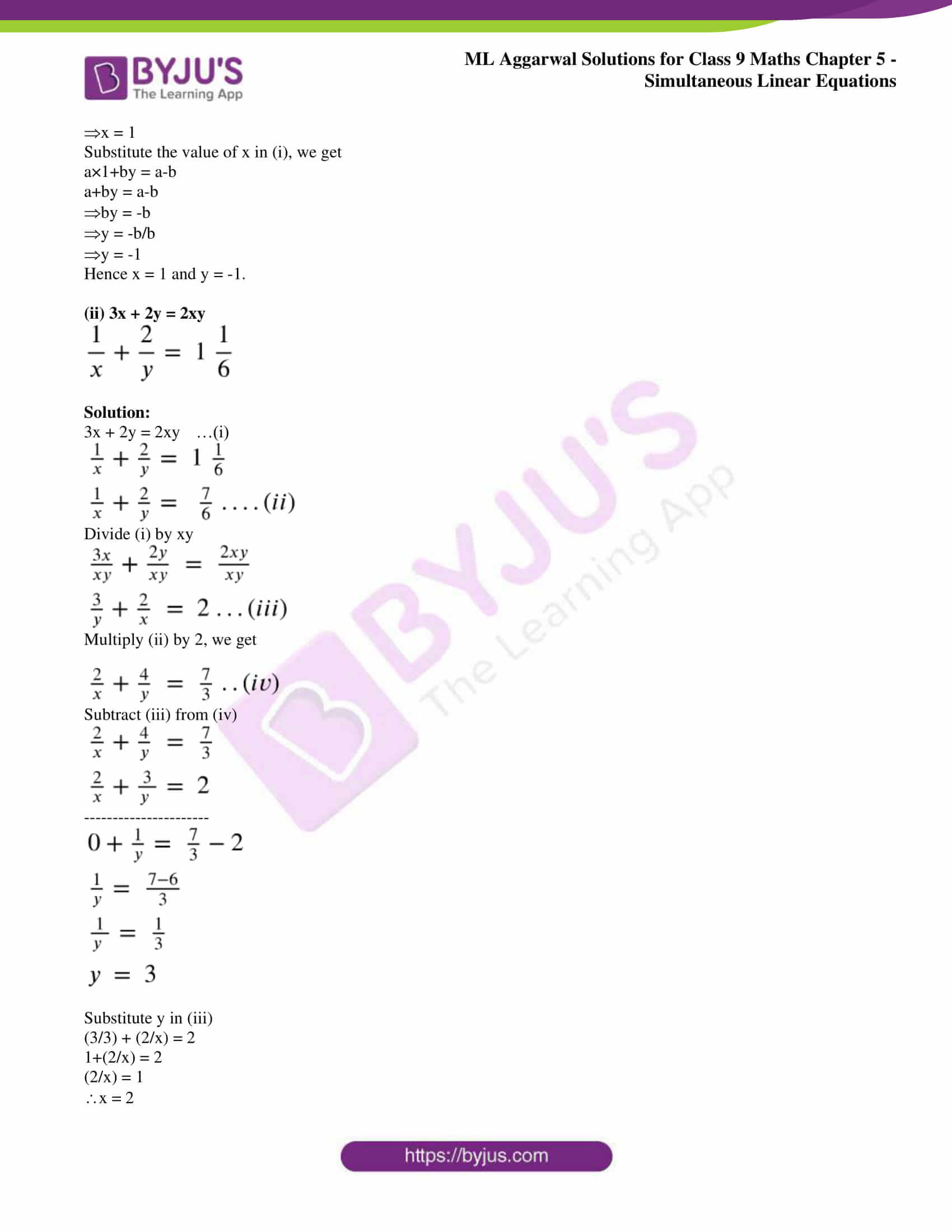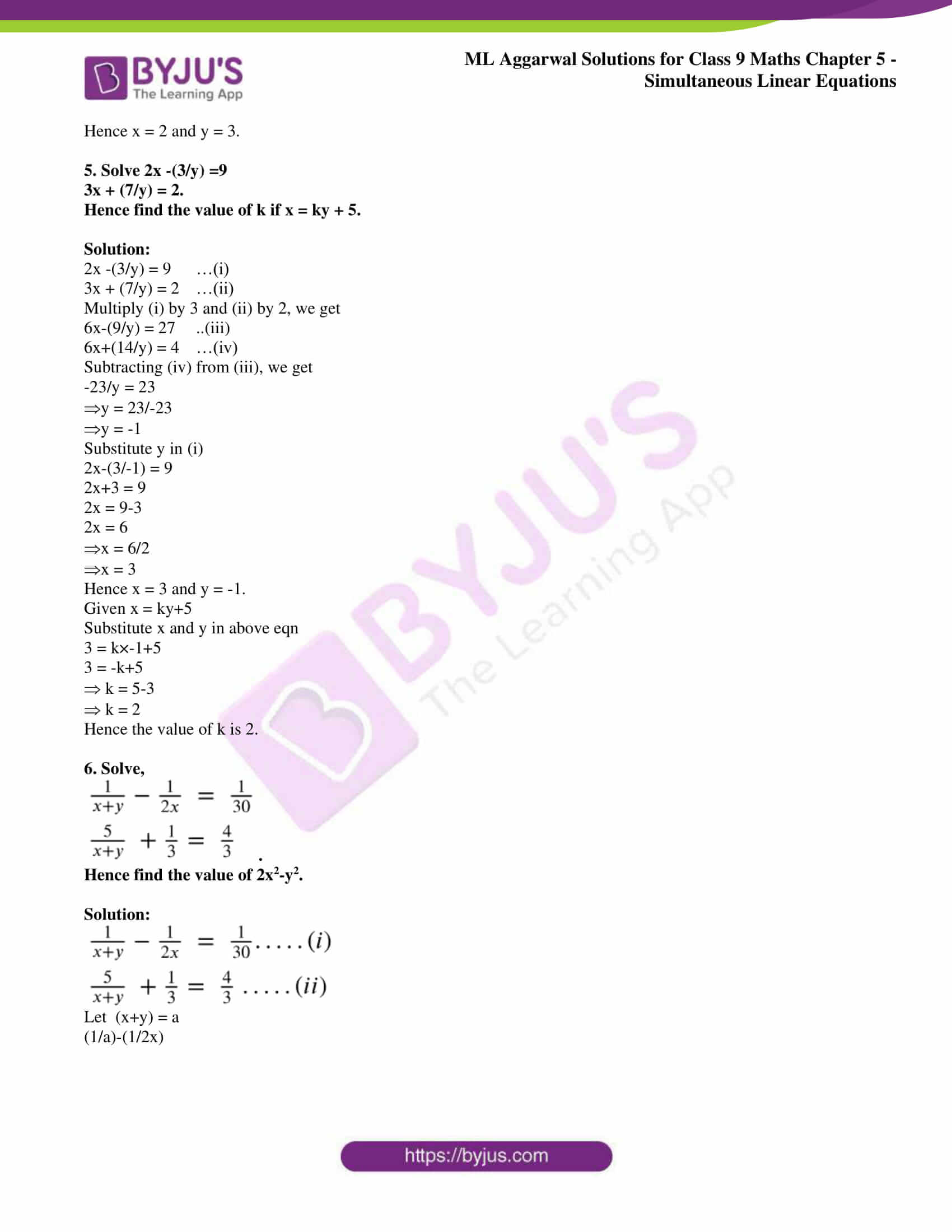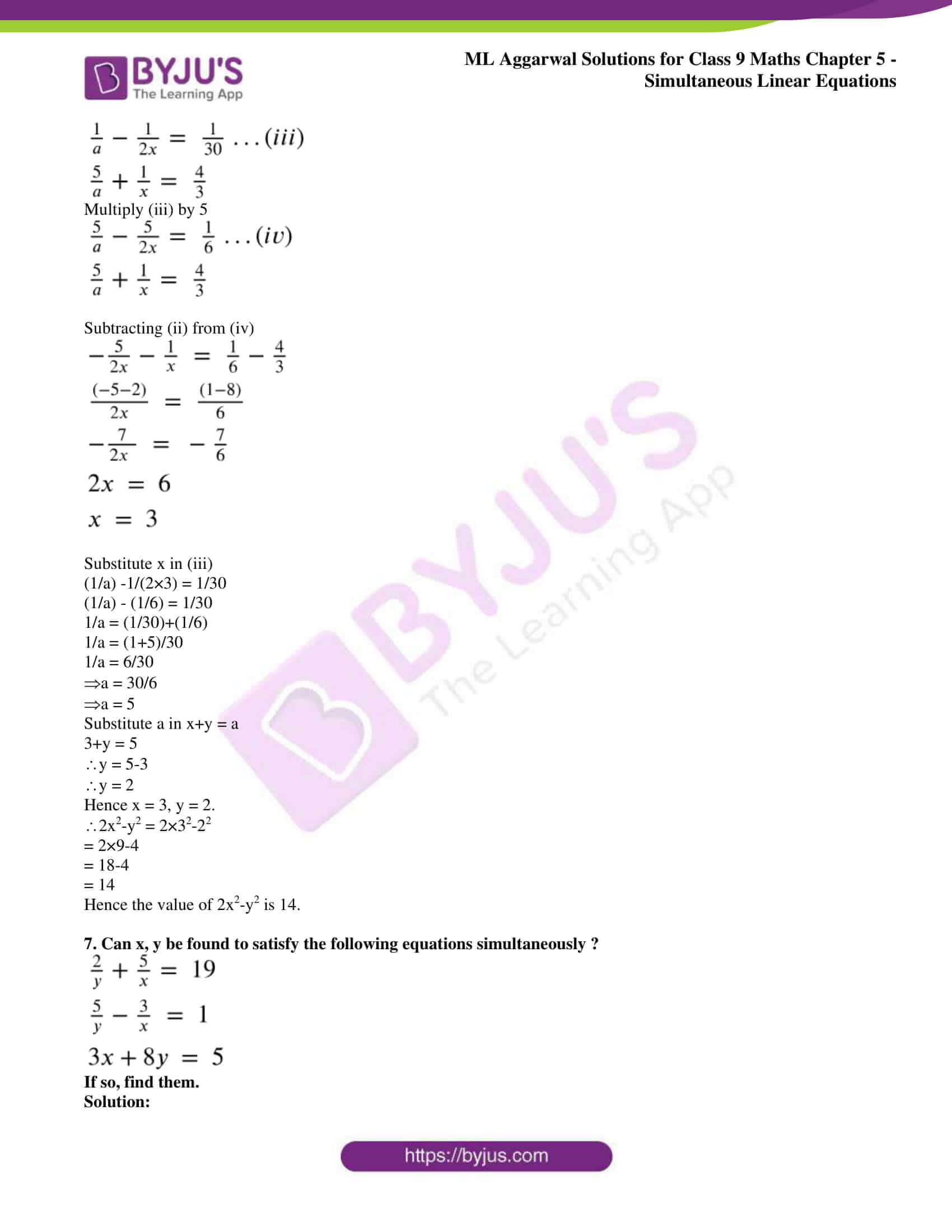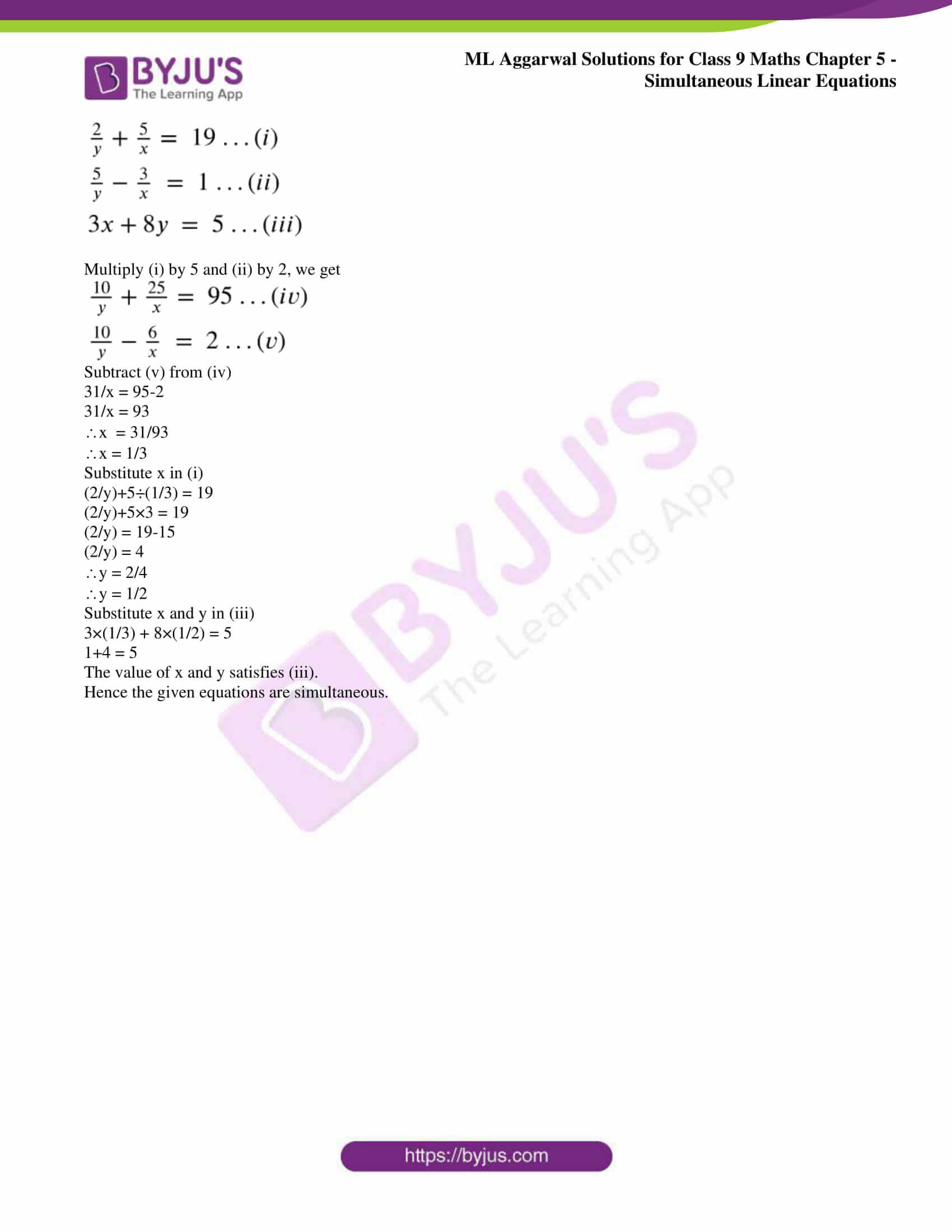### Access answers to ML Aggarwal Solutions for Class 9 Maths Chapter 5 – Simultaneous Linear Equations

Exercise 5.1

Solve the following systems of simultaneous linear equations by the substitution method (1 to 4):

1. (i) x + y = 14

x – y = 4

(ii) s – t = 3

s/3 + t/2 = 6

(iii) 2x + 3y = 9

3x + 4y = 5

(iv) 3x – 5y = 4

9x – 2y = 7

Solution:

(i) x + y = 14

x – y = 4

It can be written as

x = 4 + y

By substituting the value in the above equation

4 + y + y = 14

By further calculation

2y = 14 – 4 = 10

Dividing by 2

y = 10/2 = 5

So we get

x = 4 + 5 = 9

Hence, x = 9 and y = 5.

(ii) s – t = 3

s/3 + t/2 = 6

By taking LCM

2s + 3t = 6 × 6 = 36

We know that

s – t = 3 …. (1)

2s + 3t = 36 ….. (2)

So we get

s = 3 + t …. (3)

By substituting the value of s in equation (2)

2 (3 + t) + 3t = 36

By further calculation

6 + 2t + 3t = 36

So we get

5t = 36 – 6 = 30

By division

t = 30/5 = 6

Substituting t in equation (3)

s = 3 + 6 = 9

Hence, s = 9 and t = 6.

(iii) 2x + 3y = 9 …. (1)

3x + 4y = 5 ….. (2)

Equation (1) can be written as

2x = 9 – 3y

x = (9 – 3y)/ 2 …. (3)

By substituting the value of x in equation (2)

3 × (9 – 3y)/ 2 + 4y = 5

By further calculation

(27 – 9y)/ 2 + 4y = 5

By taking LCM

27 – 9y + 8y = 10

So we get

-y = – 17

y = 17

Substituting y in equation (3)

x = [9 – (3 × 17)]/ 2

By further calculation

x = (9 – 51)/ 2

x = – 21

Hence, x = – 21 and y = 17.

(iv) 3x – 5y = 4 ….. (1)

9x – 2y = 7 …. (2)

Multiply equation (1) by 3

9x – 15y = 12

9x – 2y = 7

By subtracting both the equations

– 13y = 5

y = -5/13

Equation (1) can be written as

3x – 5y = 4

x = (4 + 5y)/ 3 ….. (3)

By substituting the value of x in equation (2)

9 [(4 + 5y)/ 3] – 2y = 7

By further calculation

12 + 15y – 2y = 7

13y = – 5

So we get

y = -5/13

Substituting y in equation (3)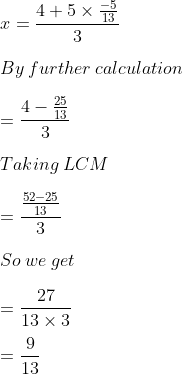Hence, x = 9/13 and y = – 5/13.

2. (i) a + 3b = 5

7a – 8b = 6

(ii) 5x + 4y – 4 = 0

x – 20 = 12y

Solution:

(i) a + 3b = 5 …. (1)

7a – 8b = 6 ….. (2)

Now multiply equation (1) by 7

7a + 21b = 35 …. (3)

7a – 8b = 6 ….. (4)

By subtracting both the equations

29b = 29

So we get

b = 29/29 = 1

Now substituting b = 1 in equation (1)

a + 3 (1) = 5

By further calculation

a + 3 = 5

So we get

a = 5 – 3 = 2

Therefore, a = 2 and b = 1.

(ii) 5x + 4y – 4 = 0

x – 20 = 12y

We can write it as

5x + 4y = 4 …. (1)

x – 12y = 20 ….. (2)

Now multiply equation (2) by 5

5x + 4y = 4 …. (3)

5x – 60y = 100

By subtracting both the equations

64y = – 96

So we get

y = -96/64 = – 3/2

Now substitute the value of y in equation (1)

5x + 4 (-3/2) = 4

By further calculation

5x + 2 (-3) = 4

So we get

5x – 6 = 4

5x = 4 + 6 = 10

By division

x = 10/5 = 2

Therefore, x = 2 and y = – 3/2.

3. (i) 2x – 3y/4 = 3

5x – 2y – 7 = 0

(ii) 2x + 3y = 23

5x – 20 = 8y

Solution:

(i) 2x – 3y/4 = 3

5x – 2y – 7 = 0

We can write it as

2x/1 – 3y/4 = 3

By taking LCM

(8x – 3y)/ 4 = 3

By cross multiplication

8x – 3y = 12 ….. (1)

5x – 2y = 7 …. (2)

Now multiply equation (1) by 2 and (2) by 3

16x – 6y = 24

15x – 6y = 21

By subtracting both the equations

x = 3

Now substituting the value of x in equation (1)

8 × 3 – 3y = 12

By further calculation

24 – 3y = 12

– 3y = 12 – 24

So we get

– 3y = – 12

y = – 12/-3 = 4

Therefore, x = 3 and y = 4.

(ii) 2x + 3y = 23

5x – 20 = 8y

We can write it as

2x + 3y = 23 …. (1)

5x – 8y = 20 …. (2)

By multiplying equation (1) by 5 and equation (2) by 2

10x + 15y = 115

10x – 16y = 40

By subtracting both the equations

31y = 75

So we get

y = 75/31 = 2 13/31

By substituting the value of y in equation (1)

2x + 3 (75/31) = 23

By further calculation

2x + 225/31 = 23

We can write it as

2x = 23/1 – 225/31

Taking LCM

2x = (713 – 225)/ 31 = 488/31

So we get

x = 488/ (31 × 2) = 244/ 31 = 7 27/31

Therefore, x = 7 27/31 and y = 2 13/31.

4. (i) mx – ny = m2 + n2

x + y = 2m

(ii) 2x/a + y/b = 2

x/a – y/b = 4

Solution:

(i) mx – ny = m2 + n2 …. (1)

x + y = 2m …. (2)

We can write it as

x = 2m – y ….. (3)

Now substitute the value of x in (1)

m (2m – y) – ny = m2 + n2

By further calculation

2m2 – my – ny = = m2 + n2

Taking out y as common

m2 – y (m + n) = n2

It can be written as

m2 – n2 – y (m + n) = 0

Expanding using formula

(m – n) (m + n) – y (m + n) = 0

Taking (m + n) as common

(m + n) [(m – n) – y] = 0

So we get

m – n – y = 0

y = m – n

From equation (3)

x = 2m – (m – n)

By further calculation

x = 2m – m + n = m + n

Hence, x = m + n and y = m – n.

(ii) 2x/a + y/b = 2 …. (1)

x/a – y/b = 4 …. (2)

3x/a = 6

So we get

x = 6a/3 = 2a

Substituting x in equation (1)

2 (2a)/ a + y/b = 2

By further calculation

4a/a + y/b = 2

So we get

4 + y/b = 2

y/b = 2 – 4 = – 2

Here

y = – 2b

Therefore, x = 2a and y = – 2b.

5. Solve 2x + y = 35, 3x + 4y = 65. Hence, find the value of x/y.

Solution:

It is given that

2x + y = 35 …. (1)

3x + 4y = 65 ….. (2)

Now multiply equation (1) by 4

8x + 4y = 140 …. (3)

3x + 4y = 65 …. (4)

By subtracting both the equations

5x = 75

x = 75/5 = 15

Now substituting the value of x in equation (1)

8 × 15 + 4y = 140

By further calculation

120 + 4y = 140

4y = 140 – 120

So we get

4y = 20

y = 20/4 = 5

Here

x/y = 15/5 = 3

Therefore, x/y = 3.

6. Solve the simultaneous equations 3x – y = 5, 4x – 3y = – 1. Hence, find p, if y = px – 3.

Solution:

It is given that

3x – y = 5 ….. (1)

4x – 3y = – 1 ….. (2)

Now multiply equation (1) by 3

9x – 3y = 15 ….. (3)

4x – 3y = – 1 ….. (4)

Subtracting equation (3) and (4)

5x = 16

x = 16/5

Substitute the value of x in equation (3)

3 × 16/5 – y = 5

By further calculation

48/5 – y = 5

48/5 – 5 = y

Taking LCM

(48 – 25)/ 5 = y

So we get

y = 23/5

We know that

y = px – 3

23/5 = p × 16/5 – 3

Substitute the value of x and y

23/5 + 3 = 16p/5

Taking LCM

(23 + 15)/ 5 = 16p/5

By further calculation

38/5 = 16p/5

So we get

16p = 38

p = 19/8

Therefore, x = 16/5, y = 23/5 and p = 19/8.

Exercise 5.2

Solve the following systems of simultaneous linear equations by the elimination method (1 to 9):

1. (i) 3x + 4y = 10

2x – 2y = 2

(ii) 2x = 5y + 4

3x – 2y + 16 = 0

Solution:

(i) 3x + 4y = 10 ….. (1)

2x – 2y = 2 ….. (2)

Multiplying equation (1) by 1 and (2) by 2

3x + 4y = 10

4x – 4y = 4

7x = 14

By division

x = 14/7 = 2

Substituting the value of x in equation (2)

2 × 2 – 2y = 2

By further calculation

4 – 2y = 2

So we get

2y = 4 – 2 = 2

y = 2/2 = 1

Therefore, x = 2 and y = 1.

(ii) 2x = 5y + 4

3x – 2y + 16 = 0

We can write it as

2x – 5y = 4 …. (1)

3x – 2y = – 16 ….. (2)

Now multiply equation (1) by 3 and (2) by 2

6x – 15y = 12 ….. (3)

6x – 4y = – 32 ….. (4)

By subtracting both the equations

– 11y = 44

y = -44/11 = – 4

Substitute the value of y in equation (1)

2x – 5 (-4) = 4

By further calculation

2x + 20 = 4

So we get

2x = 4 – 20 = – 16

x = – 16/2 = – 8

Therefore, x = – 8 and y = – 4.

2. (i) ¾ x – 2/3 y = 1

3/8 x – 1/6 y = 1

(ii) 2x – 3y – 3 = 0

2x/3 + 4y + ½ = 0.

Solution:

(i) ¾ x – 2/3 y = 1

3/8 x – 1/6 y = 1

We can write it as

¾ x – 2/3 y = 1

(9x – 8y)/ 12 = 1

By cross multiplication

9x – 8y = 12 ….. (1)

3/8 x – 1/6 y = 1

(9x – 4y)/ 24 = 1

By cross multiplication

9x – 4y = 24 ….. (2)

Subtracting equations (1) and (2)

– 4y = – 12

By division

y = – 12/ – 4 = 3

Substitute the value of y in (1)

9x – 8 × 3 = 12

By further calculation

9x – 24 = 12

9x = 12 + 24 = 36

By division

x = 36/ 9 = 4

Therefore, x = 4 and y = 3.

(ii) 2x – 3y – 3 = 0

2x/3 + 4y + ½ = 0

We can write it as

2x – 3y – 3 = 0

2x – 3y = 3 ….. (1)

2x/3 + 4y + ½ = 0

2x/3 + 4y = – ½

Taking LCM

(2x + 12y)/ 3 = – ½

By cross multiplication

2 (2x + 12y) = – 1 × 3

So we get

4x + 24y = – 3 …. (2)

Multiply equation (1) by 2

4x – 6y = 6

4x + 24y = – 3

By subtracting both the equations

– 30y = 9

So we get

y = -9/30 = – 3/10

Substitute the value of y in equation (1)

2x – 3 (-3/10) = 3

By further calculation

2x + 9/10 = 3

We can write it as

2x = 3 – 9/10

By taking LCM

2x = (30 – 9)/ 10

So we get

2x = 21/10

x = 21/20

Therefore, x = 21/20 and y = – 3/10.

3. (i) 15x – 14y = 117

14x – 15y = 115

(ii) 41x + 53y = 135

53x + 41y = 147.

Solution:

(i) 15x – 14y = 117 ….. (1)

14x – 15y = 115 ….. (2)

Now multiply equation (1) by 14 and (2) by 15

210x – 196y = 1638 …. (3)

210x – 225y = 1725 ….. (4)

By subtracting both the equations

29y = – 87

So we get

y = -87/29 = – 3

Substitute the value of y in equation (1)

15x – 14 (-3) = 117

By further calculation

15x + 42 = 117

So we get

15x = 117 – 42 = 75

By division

x = 75/15 = 5

Therefore, x = 5 and y = – 3.

(ii) 41x + 53y = 135 ….. (1)

53x + 41y = 147 ….. (2)

Now multiply equation (1) by 53 and (2) by 41

2173x + 2809y = 7155 ….. (3)

2173x + 1681y = 6027 ….. (4)

By subtracting both the equations

1128y = 1128

So we get

y = 1128/1128 = 1

Substitute the value of y in equation (1)

41x + 53 × 1 = 135

By further calculation

41x + 53 = 135

So we get

41x = 135 – 53 = 82

By division

x = 82/41 = 2

Therefore, x = 2 and y = 1.

4. (i) x/6 = y – 6

3x/4 = 1 + y

(ii) x – 2/3 y = 8/3

2x/5 – y = 7/5.

Solution:

(i) x/6 = y – 6

3x/4 = 1 + y

We can write it as

x = 6 (y – 6)

x = 6y – 36

x – 6y = – 36 ….. (1)

3x/4 = 1 + y

By cross multiplication

3x = 4 (1 + y)

So we get

3x = 4 + 4y

3x – 4y = 4 …. (2)

Multiply equation (1) by 3

3x – 18y = – 108

3x – 4y = 4

Subtracting both the equations

– 14y = – 112

So we get

y = – 112/- 14 = 8

Substitute the value of y in equation (1)

x – 6 × 8 = – 36

By further calculation

x – 48 = – 36

x = – 36 + 48

x = 12

Therefore, x = 12 and y = 8.

(ii) x – 2/3 y = 8/3

2x/5 – y = 7/5

We can write it as

x – 2/3 y = 8/3

Taking LCM

(3x – 2y)/ 3 = 8/3

By cross multiplication

3x – 2y = 8/3 × 3 = 8

3x – 2y = 8 ….. (1)

2x/5 – y = 7/5

Taking LCM

(2x – 5y)/ 5 = 7/5

By cross multiplication

2x – 5y = 7/5 × 5 = 7

2x – 5y = 7 …… (2)

Multiply equation (1) by 2 and (2) by 3

6x – 4y = 16 ….. (3)

6x – 15y = 21 …… (4)

Subtracting both the equations

11y = – 5

y = – 5/11

Substitute the value of y in equation (1)

3x – 2 (-5/11) = 8

By further calculation

3x + 10/11 = 8

We can write it as

3x = 8 – 10/11

Taking LCM

3x = (88 – 10)/ 11 = 78/11

By cross multiplication

x = 78/ (11 × 3) = 26/11

Therefore, x = 26/11 and y = – 5/11.

5. (i) 9 – (x – 4) = y + 7

2 (x + y) = 4 – 3y

(ii) 2x + (x – y)/ 6 = 2

x – (2x + y)/3 = 1.

Solution:

(i) 9 – (x – 4) = y + 7

2 (x + y) = 4 – 3y

We can write it as

9 – (x – 4) = y + 7

9 – x + 4 = y + 7

By further calculation

13 – x = y + 7

– x – y = 7 – 13 = – 6

x + y = 6 ….. (1)

2 (x + y) = 4 – 3y

2x + 2y = 4 – 3y

By further calculation

2x + 2y + 3y = 4

So we get

2x + 5y = 4 ….. (2)

Now multiply equation (1) by 5 and (2) by 1

5x + 5y = 30

2x + 5y = 4

By subtracting both the equations

3x = 26

So we get

x = 26/3

Substitute the value of x in (1)

26/3 + y = 6

We can write it as

y = 6 – 26/3

Taking LCM

y = (18 – 26)/3

So we get

y = – 8/3

Therefore, x = 26/3 and y = – 8/3.

(ii) 2x + (x – y)/ 6 = 2

x – (2x + y)/3 = 1

2x + (x – y)/ 6 = 2

Multiply by 6

12x + x – y = 12

By further calculation

13x – y = 12 …… (2)

x – (2x + y)/3 = 1

Multiply by 3

3x – 2x – y = 3

By further calculation

x – y = 3 …… (2)

So we get

x = 3 + y ….. (3)

Substitute the value of x in (1)

13 (3 + y) – y = 12

By further calculation

39 + 13y – y = 12

So we get

12y = 12 – 39 = – 27

By division

y = – 27/12 = – 9/4

Substitute the value of y in (3)

x = 3 + y

x = 3 + (-9)/4

By further calculation

x = 3 – 9/4

Taking LCM

x = (12 – 9)/ 4

x = ¾

Therefore, x = ¾ and y = – 9/4.

6. x – 3y = 3x – 1 = 2x – y.

Solution:

It is given that

x – 3y = 3x – 1 = 2x – y

Here

x- 3y = 3x – 1

x – 3x – 3y = – 1

By further calculation

– 2x – 3y = – 1

2x + 3y = 1 ….. (1)

3x – 1 = 2x – y

3x – 2x + y = 1

By further simplification

x + y = 1 ….. (2)

Multiply equation (2) by 2 and subtract from equation (1)

2x + 3y = 1

2x + 2y = 2

So we get

y = – 1

Substitute the value of y in equation (1)

2x + 3 (-1) = 1

So we get

2x – 3 = 1

2x = 1 + 3 = 4

By division

x = 4/2 = 2

Therefore, x = 2 and y = – 1.

7. (i) 4x + (x – y)/ 8 = 17

2y + x – (5y + 2)/3 = 2

(ii) (x + 1)/2 + (y – 1)/3 = 8

(x – 1)/3 + (y + 1)/ 2 = 9.

Solution:

(i) 4x + (x – y)/ 8 = 17

2y + x – (5y + 2)/3 = 2

We can write it as

4x + (x – y)/ 8 = 17

(32 + x – y)/ 8 = 17

By further calculation

(33x – y)/ 8 = 17

By cross multiplication

33x – y = 136 ….. (1)

2y + x – (5y + 2)/3 = 2

Taking LCM

[3 (2y + x) – 5 (5y + 2)]/ 3 = 2

By further calculation

6y + 3x – 5y – 2 = 2 × 3

So we get

y + 3x – 2 = 6

3x + y = 6 + 2

3x + y = 8 ….. (2)

36x = 144

By division

x = 144/36 = 4

Substitute the value of x in equation (1)

33 × 4 – y = 136

By further calculation

132 – y = 136

– y = 136 – 132

So we get

– y = 4

y = – 4

Therefore, x = 4 and y = – 4.

(ii) (x + 1)/2 + (y – 1)/3 = 8

(x – 1)/3 + (y + 1)/ 2 = 9

We can write it as

(x + 1)/2 + (y – 1)/3 = 8

Taking LCM

(3x + 3 + 2y – 2)/6 = 8

By further calculation

3x + 2y + 1 = 48

So we get

3x + 2y = 47 ….. (1)

(x – 1)/3 + (y + 1)/ 2 = 9

Taking LCM

(2x – 2 + 3y + 3)/6 = 9

By further calculation

2x + 3y + 1 = 54

So we get

2x + 3y = 53 ….. (2)

By adding equation (1) and (2)

5x + 5y = 100

Dividing by 5

x + y = 20 …… (3)

By subtracting equation (1) and (2)

x – y = – 6 ….. (4)

Now add equation (3) and (4)

2x = 14

x = 14/2 = 7

Subtracting equation (4) and (3)

2y = 26

y = 26/2 = 13

Therefore, x = 7 and y = 13.

8. (i) 3/x + 4y = 7

5/x + 6y = 13

(ii) 5x – 9 = 1/y

x + 1/y = 3.

Solution:

(i) 3/x + 4y = 7 ….. (1)

5/x + 6y = 13 ….. (2)

Substitute 1/x = a in equation (1) and )@)

3a + 4y = 7 …. (3)

5a + 6y = 13 ….. (4)

Multiply equation (3) by 5 and (4) by 3

15a + 20y = 35

15a + 18y = 39

Subtracting both the equations

2y = -4

So we get

y = – 4/2 = – 2

Substitute the value of y in equation (3)

3a + 4 (-2) = 7

By further calculation

3a – 8 = 7

3a = 7 + 8 = 15

So we get

3a = 15

a = 15/3 = 5

Here x = 1/a = 1/5

Therefore, x = 1/5 and y = – 2.

(ii) 5x – 9 = 1/y ….. (1)

x + 1/y = 3 ….. (2)

Substitute 1/y = b in (1) and (2)

5x – 9 = b

5x – b = 9 ….. (3)

x + b = 3 ….. (4)

By adding equation (3) and (4)

5x – b = 9 ….. (3)

x + b = 3 ….. (4)

So we get

6x = 12

By division

x = 12/6 = 2

Substitute the value of x in equation (4)

2 + b = 3

b = 3 – 2

b = 1

Here 1/y = 1

b = 1/y

y = 1

Therefore, x = 2 and y = 1.

9. (i) px + qy = p – q

qx – py = p + q

(ii) x/a – y/b = 0

ax + by = a2 + b2.

Solution:

(i) px + qy = p – q …. (1)

qx – py = p + q ….. (2)

Now multiply equation (1) by p and (2) by q

p2x + pqy = p2 – pq

q2x – pqy = pq + q2

(p2 + q2) x = p2 + q2

By further calculation

x = (p2 + q2)/ (p2 + q2) = 1

From equation (1)

p × 1 + qy = p – q

By further calculation

p – qy = p – q

So we get

qy = p – q – p = – q

Here

y = -q/q = – 1

Therefore, x = 1 and y = – 1.

(ii) x/a – y/b = 0

ax + by = a2 + b2

We can write it as

x/a – y/b = 0

Taking LCM

(bx – ay)/ab = 0

By cross multiplication

bx – ay = 0 …… (1)

ax + by = a2 + b2 ….. (2)

Multiply equation (1) by b and equation (2) by a

b2x – aby = 0

a2x + aby = a2 + ab2

(a2 + b2)x = a2+ ab2 = a (a2 + b2)

So we get

x = a (a2 + b2)/ a2 + b2 = a

From equation (2)

b × a – ay = 0

By further calculation

ab – ay = 0

ay = ab

So we get

y = ab/a = b

Therefore, x = a and y = b.

10. Solve 2x + y = 23, 4x – y = 19. Hence, find the values of x – 3y and 5y – 2x.

Solution:

It is given that

2x + y = 23 …. (1)

4x – y = 19 ….. (2)

6x = 42

x = 42/6 = 7

Substitute the value of x in equation (1)

2 × 7 + y = 23

By further calculation

14 + y = 23

So we get

y = 23 – 14 = 9

Therefore, x = 7 and y = 9.

x – 3y = 7 – 3 × 9 = 7 – 27 = – 20

5y – 2x = 5 × 9 – 2 × 7 = 45 – 14 = 31

11. The expression ax + by has value 7 when x = 2, y = 1. When x = – 1, y = 1, it has value 1, find a and b.

Solution:

It is given that

ax + by = 7 when x = 2 and y = 1

Substituting the values

a (2) + b (1) = 7

2a + b = 7 ….. (1)

Here

ax + by = 1 when x = – 1 and y = 1

Substituting the values

a (-1) + b (1) = 1

– a + b = 1 ….. (2)

By subtracting both the equations

– 3a = – 6

So we get

a = – 6/ – 3 = 2

Substituting the value of a in equation (1)

2 × 2 + b = 7

By further calculation

4 + b = 7

b = 7 – 4 = 3

Therefore, a = 2 and b = 3.

12. Can the following equations hold simultaneously?

3x – 7y = 7

11x + 5y = 87

5x + 4y = 43.

If so, find x and y.

Solution:

3x – 7y = 7 …… (1)

11x + 5y = 87 ….. (2)

5x + 4y = 43 ….. (3)

Now multiply equation (1) by 5 and (2) by 7

15x – 35y = 35

77x + 35y = 609

92x = 644

By division

x = 644/92 = 7

Substitute the value of x in equation (1)

3 × 7 – 7y = 7

By further calculation

21 – 7y = 7

So we get

– 7y = 7 – 21 = – 14

y = – 14/ – 7 = 2

Therefore, x = 7 and y = 2.

If x = 7 and y – 2 satisfy the equation (3) then we can say that the equations hold simultaneously

Substitute the value of x and y in equation (3)

5x + 4y = 43

By further calculation

5 × 7 + 4 × 2 = 43

So we get

35 + 8 = 43

43 = 43 which is true.

Therefore, the equations hold simultaneously.

Exercise 5.3

1. Solve the following systems of simultaneous linear equations by cross-multiplication method:

(i) 3x + 2y = 4

8x + 5y = 9

(ii) 3x – 7y + 10 = 0

y – 2x = 3.

Solution:

(i) 3x + 2y = 4

8x + 5y = 9

We can write it as

3x + 2y – 4 = 0

8x + 4y – 9 = 0

By cross multiplication method

x/ (-18 + 20) = y/ (-32 + 27) = 1/ (15 – 16)

By further calculation

x/2 = y/-5 = 1/-1

So we get

x/2 = – 1

x = – 2

y = – 5 (-1) = 5

Therefore, x = – 2 and y = 5.

(ii) 3x – 7y + 10 = 0

y – 2x = 3

We can write it as

3x – 7y + 10 = 0

y – 2x – 3 = 0

By cross multiplication method

x/ (21 – 10) = y/ (-20 + 9) = 1/ (3 – 14)

By further calculation

x/11 = y/-11 = 1/-11

So we get

x/11 = 1/-11

x = – 1

Similarly

y/-11 = 1/ -11

y = 1

Therefore, x = – 1 and y = 1.

2. Solve the following pairs of linear equations by cross-multiplication method:

(i) x – y = a + b

ax + by = a2 – b2

(ii) 2bx + ay = 2ab

bx – ay = 4ab.

Solution:

(i) x – y = a + b

ax + by = a2 – b2

We can write it as

x – y – (a + b) = 0

ax + by – (a2 – b2) = 0

By cross multiplication method

x/ [a2 – b2 + b (a + b)] = y/ [- a (a + b) + a2 – b2] = 1/ (b + a)

By further calculation

x/ (a2 – b2 + ab + b2) = y/ (-a2 – ab + a2 – b2) = 1/ (a + b)

So we get

x/ [a (a + b)] = y/ [-b (a + b)] = 1/ (a + b)

x = a (a + b)/ (a + b) = a

y = [-b (a + b)]/ (a + b) = – b

Therefore, x = a and y = – b.

(ii) 2bx + ay = 2ab

bx – ay = 4ab

We can write it as

2bx + ay – 2an = 0

bx – ay – 4ab = 0

By cross multiplication method

x/ (- 4a2b – 2a2b) = y/ (-2ab2 + 8ab2) = 1/ (-2ab – ab)

By further calculation

x/ -6a2b = y/6ab2= 1/-3ab

So we get

x = -6a2b/ -3ab = 2a

y = 6ab2/-3ab = – 2b

Therefore, x = 2a and b = – 2b.

Exercise 5.4

Solve the following pairs of linear equations (1 to 5):

1. (i) 2/x + 2/3y = 1/6

2/x – 1/y = 1

(ii) 3/2x + 2/3y = 5

5/x – 3/y = 1.

Solution:

(i) 2/x + 2/3y = 1/6 ….. (1)

2/x – 1/y = 1 ….. (2)

By subtracting both the equations

5/3y = -5/6

By cross multiplication

– 15y = 30

By division

y = 30/ -15 = – 2

Substitute the value of y in equation (1)

2/x + 2/ (3 × (-2)) = 1/6

By further calculation

2/x – 1/3 = 1/6

So we get

2/x = 1/6 + 1/3

Taking LCM

2/x = (1 + 2)/ 6 = 3/6

By cross multiplication

x = (2 × 6)/3 = 12/3 = 4

Therefore, x = 4 and y = – 2.

(ii) 3/2x + 2/3y = 5 ….. (1)

5/x – 3/y = 1 ….. (2)

Multiply equation (1) by 1 and (2) by 2/9

3/2x + 2/3y = 5

10/9x – 2/3y = 2/9

(3/2 + 10/9)1/x = 5 + 2/9

Taking LCM

(27 + 20)/ 18 × 1/x = (45 + 2)/ 9

By further calculation

47/18x = 47/9

By cross multiplication

x = (47 × 9)/ (47 × 18) = ½

Substitute the value of x in equation (2)

5/ ½ – 3/y = 1

By further calculation

10 – 3/y = 1

3/y = 10 – 1 = 9

So we get

y = 3/9 = 1/3

Therefore, x = ½ and y = 1/3.

2. (i) (7x – 2y)/ xy = 5

(8x + 7y)/ xy = 15

(ii) 99x + 101y = 499xy

101x + 99y = 501xy.

Solution:

(i) (7x – 2y)/ xy = 5

(8x + 7y)/ xy = 15

We can write it as

7x/xy – 2y/xy = 5

8x/xy + 7y/xy = 15

By further simplification

7/y – 2/x = 5 …. (1)

8/y + 7/x = 15 ….. (2)

Now multiply equation (1) by 7 and (2) by 2

49/y – 14/x = 35

16/y + 14/x = 30

65/y = 65

So we get

y = 65/65 = 1

Substitute the value of y in equation (1)

7/1 – 2/x = 5

By further calculation

2/x = 7 – 5 = 2

So we get

x = 2/2 = 1

Therefore, x = 1 and y = 1.

(ii) 99x + 101y = 499xy

101x + 99y = 501xy

Now divide each term by xy

99x/xy + 101y/xy = 499xy/xy

101y/xy + 99x/xy = 501xy/xy

By further calculation

99/y + 101/x = 499 ….. (1)

101/y + 99/x = 501 ….. (2)

200/y + 200/x = 1000

Divide by 200

1/y + 1/x = 5 …… (3)

Subtracting both the equations

-2/y + 2/x = – 2

Divide by 2

-1/y + 1/x = – 1 …. (4)

By adding equation (3) and (4)

2/x = 4

So we get

x = 2/4 = ½

By subtracting equation (3) and (4)

2/y = 6

So we get

y = 2/6 = 1/3

Therefore, x = ½ and y = 1/3 if x ≠ 0, y ≠ 0.

3. (i) 3x + 14y = 5xy

21y – x = 2xy

(ii) 3x + 5y = 4xy

2y – x = xy.

Solution:

(i) 3x + 14y = 5xy

21y – x = 2xy

Now dividing each equation by xy of x ≠ 0, y ≠ 0

3x/xy + 14y/xy = 5xy/xy

By further calculation

3/y = 14/x = 5 ….. (1)

(ii) 3x + 5y = 4xy

2y – x = xy

We can write it as

3x + 5y = 4xy

– x + 2y = xy

Divide each equation by xy if x≠ 0 and y ≠ 0

3x/xy + 5y/xy = 4xy/xy

So we get

3/y + 5/x = 4 ….. (1)

-x/xy + 2y/xy = xy/xy

So we get

-1/y + 2/x = 1 ….. (2)

Now multiply equation (1) by 1 and (2) by 3

3/y + 5/x = 4

-3/y + 6/x = 3

11/x = 7

So we get

x = 11/7

Substitute the value of x in equation (2)

-1/y + 2/11/7 = 1

By further calculation

-1/y + (2 × 7)/ 11 = 1

-1/y + 14/11 = 1

We can write it as

-1/y = 1 – 14/11

Taking LCM

-1/y = (11 – 14)/ 11

So we get

-1/y = -3/11

By cross multiplication

-3y = – 11

y = – 11/-3 = 11/3

Therefore, x = 11/7 and y = 11/3.

4. (i) 20/ (x + 1) + 4/ (y – 1) = 5

10/ (x + 1) – 4/ (y – 1) = 1

(ii) 3/ (x + y) + 2/ (x – y) = 3

2/ (x + y) + 3/ (x – y) = 11/3.

Solution:

(i) 20/ (x + 1) + 4/ (y – 1) = 5 ….. (1)

10/ (x + 1) – 4/ (y – 1) = 1 ….. (2)

30/ (x + 1) = 6

By cross multiplication

30 = 6 (x + 1)

By further calculation

30/6 = x + 1

5 = x + 1

So we get

x = 5 – 1 = 4

Substitute the value of x in equation (1)

20/ (x + 1) + 4/ (y – 1) = 5

20/ (4 + 1) + 4/ (y – 1) = 5

By further calculation

20/5 + 4/ (y – 1) = 5

4 + 4/ (y – 1) = 5

We can write it as

4/ (y – 1) = 5 – 4 = 1

4/ (y – 1) = 1

By cross multiplication

4 = 1 (y – 1)

So we get

4 = y – 1

y = 4 + 1 = 5

Therefore, x = 4 and y = 5.

(ii) 3/ (x + y) + 2/ (x – y) = 3 …. (1)

2/ (x + y) + 3/ (x – y) = 11/3 ….. (2)

Multiply equation (1) by 3 and (2) by 2

9/ (x + y) + 6/ (x – y) = 9 ….. (3)

4/ (x + y) + 6/ (x – y) = 22/3 ….. (4)

Subtracting both the equations

5/ (x + y) = 9 – 22/3

Taking LCM

5/ (x + y) = 5/3

By cross multiplication

5 × 3 = 5 (x + y)

By further calculation

(5 × 3)/ 5 = x + y

x + y = (3 × 1)/ 3

x + y = 3 …… (5)

Substitute equation (5) in (1)

3/3 + 2/ (x – y) = 3

By further calculation

1 + 2/ (x – y) = 3

2/ (x – y) = 3 – 1 = 2

So we get

2/2 = x – y

Here

1 = x – y ….. (6)

We can write it as

x – y = 1

x + y = 3

2x = 4

x = 4/2 = 2

Substitute x = 2 in equation (5)

2 + y = 3

y = 3 – 2 = 1

Therefore, x = 2 and y = 1.

5. (i) 1/ 2(2x + 3y) + 12/ 7(3x – 2y) = ½

7/ (2x + 3y) + 4/ (3x – 2y) = 2

(ii) 1/ 2(x + 2y) + 5/ 3(3x – 2y) = – 3/2

5/ 4(x + 2y) – 3/ 5 (3x – 2y) = 61/60.

Solution:

(i) 1/ 2(2x + 3y) + 12/ 7(3x – 2y) = ½

7/ (2x + 3y) + 4/ (3x – 2y) = 2

Consider 2x + 3y = a and 3x – 2y = b

We can write it as

1/2a + 12/7b = ½

7/a + 4/b = 2

Now multiply equation (1) by 7 and (2) by ½

7/2a + 12/b = 7/2

7/2a + 2/b = 1

Subtracting both the equations

10/b = 5/2

So we get

b = (10 × 2)/ 5 = 4

Substitute the value of b in equation (2)

7/a + 4/4 = 2

7/a + 1 = 2

So we get

7/a = 2 – 1 = 1

a = 7

Here

2x + 3y = 7 ….. (3)

3x – 2y = 4 ….. (4)

Multiply equation (3) by 2 and (4) by 3

4x + 6y = 14

9x – 6y = 12

So we get

13x = 26

x = 26/13 = 2

Substitute the value of x in (3)

2 × 2 + 3y = 7

By further calculation

4 + 3y = 7

So we get

3y = 7 – 4 = 3

y = 3/3 = 1

Therefore, x = 2 and y = 1.

(ii) 1/ 2(x + 2y) + 5/ 3(3x – 2y) = – 3/2

5/ 4(x + 2y) – 3/ 5 (3x – 2y) = 61/60

Consider x + 2y = a and 3x – 2y = b

1/2a + 5/3b = – 3/2 …. (1)

5/4a – 3/5b = 61/60 ….. (2)

Now multiply equation (1) by 5/2 and (2) by (1)

5/4a + 25/6b = – 15/4

5/4a – 3/5b = 61/60

Subtracting both the equations

25/6b + 3/5b = – 15/4 – 61/60

Taking LCM

(125 + 18)/ 30b = (-225 – 61)/ 60

By further calculation

143/30b = – 286/60

By cross multiplication

30b × (-286) = 60 × 143

So we get

b = (60 × 143)/ (30 × -286) = – 1

Substitute the value of b in equation (1)

1/2a + 5/ (3 × – 1) = -3/2

By further calculation

1/2a – 5/4 = – 3/2

We can write it as

1/2a = – 3/2 + 5/3

Taking LCM

1/2a = (-9+ 10)/ 6 = 1/6

So we get

a = 6/2 = 3

Here

x + 2y = 3 ….. (3)

3x – 2y = – 1 ….. (4)

4x = 2

x = 2/4 = ½

Substitute the value of x in equation (3)

½ + 2y = 3

By further calculation

2y = 3 – ½

Taking LCM

2y = 5/2

y = 5/ (2 × 2) = 5/4

Therefore, x = ½ and y = 5/4.

Chapter Test

Solve the following simultaneous linear equations (1 to 4):

1.(i) 2x – (¾)y = 3,

5x – 2y = 7

Solution:8x-3y = 12 …(i)

5x-2y = 7 …(ii)

Multiply (i) by 5 and (ii) by 8, we get

40x-15y = 60 (iii)

40x -16y = 56 (iv)

Subtract (iv) from (iii), we get

y = 4

Substitute y in (i)

8x-3×4 = 12

8x = 12+12

8x = 24

x = 24/8

x = 3

Hence x = 3 and y = 4.

(ii) 2(x-4) = 9y+2

x – 6y = 2

Solution:

2(x-4) = 9y+2

2x-8 = 9y+2

2x-9y = 2+8

2x-9y = 10 ….(i)

x-6y = 2 ….(ii)

Multiply (ii) by 2, we get

2x -12y = 4 ….(iii)

Subtract (iii) from (i), we get

2x-9y = 10

-2x +12y = -4

——————

0+3y = 6

3y = 6

y = 6/3

y = 2

Substitute the value of y in (i)

2x-9×2 = 10

2x-18 = 10

2x = 10+18

2x = 28

x = 28/2

x = 14

Hence x = 14 and y = 2.

2. (i) 97x+53y = 177

53x+97y = 573

Solution:

Given equations are as follows.

97x+53y = 177 …(i)

53x+97y = 573 …(ii)

Multiply (i) by 53 and (ii) by 97

53(97x+53y) = 53×177

5141x+2809y = 9381 …..(iii)

97(53x+97y) = 97×573

5141x+9409y = 55581 …..(iv)

Subtract (iv) from (iii)

5141x+2809y = 9381 …..(iii)

5141x+9409y = 55581 …..(iv)

——————————

0x -6600y = -46200

-6600y = -46200

y = -46200/-6600

y = 7

Substitute the value of y in (i)

97x+53×7 = 177

97x+371 = 177

97x = 177-371

97x = – 194

x = -194/97

x = -2

Hence x = -2 and y = 7.

(ii) x+y = 5.5

x-y = 0.9

Solution:

x+y = 5.5 …(i)

x-y = 0.9 …(ii)

——————–

Adding (i) and (ii), we get

2x = 5.5+0.9

2x = 6.4

x = 6.4/2

x = 3.2

Substitute value of x in (i)

3.2+y = 5.5

y = 5.5-3.2

y = 2.3

Hence x = 3.2 and y = 2.3.

3. (i) x+y = 7xy

2x-3y+xy = 0

Solution:

x+y = 7xy …(i)

2x-3y+xy = 0 …..(ii)

Divide (i) by xy, we get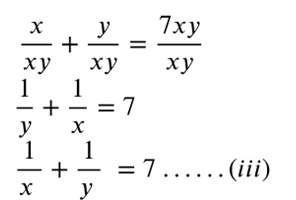Divide (ii) by xy, we get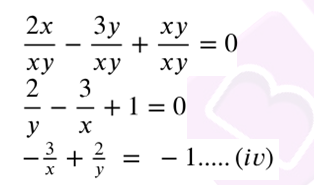Multiplying (iii) by 3, we get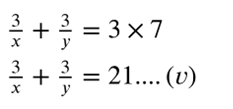Adding (v) and (iv), we get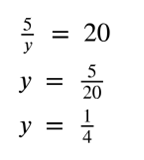Substitute value of y in (iv)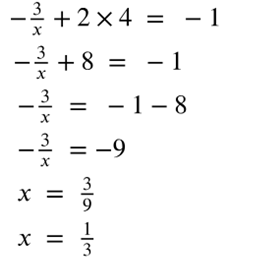Hence x = 1/3 and y = 1/4.

(ii)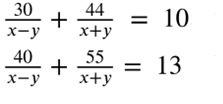Solution:Multiply (i) by 4 and (ii) by 3, we getSubtracting (iv) from (iii), we getSubstitute (v) in (i), we getNow solve for (v) and (vi)

x+y = 11

x-y = 5

2x = 16

x = 16/2 = 8

Substitute x in (v)

8+y = 11

y = 11-8

y = 3

Hence x = 8 and y = 3.

4. (i) ax+by = a-b

bx-ay = a+b

Solution:

ax+by = a-b …(i)

bx-ay = a+b …(ii)

multiplying (i) by a and (ii) by b, we get

a(ax+by) = a(a-b)

a2x +aby = a2-ab …(iii)

b(bx-ay) = b(a+b)

b2x -aby = ab+b2 …(iv)

a2x +aby = a2-ab

b2x -aby = ab+b2

———————–

(a2+b2)x = (a2+b2)

x = (a2+b2)/ (a2+b2)

x = 1

Substitute the value of x in (i), we get

a×1+by = a-b

a+by = a-b

by = -b

y = -b/b

y = -1

Hence x = 1 and y = -1.

(ii) 3x + 2y = 2xySolution:

3x + 2y = 2xy …(i)Divide (i) by xyMultiply (ii) by 2, we get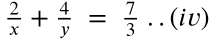Subtract (iii) from (iv)———————-Substitute y in (iii)

(3/3) + (2/x) = 2

1+(2/x) = 2

(2/x) = 1

x = 2

Hence x = 2 and y = 3.

5. Solve 2x -(3/y) =9

3x + (7/y) = 2.

Hence find the value of k if x = ky + 5.

Solution:

2x -(3/y) = 9 …(i)

3x + (7/y) = 2 …(ii)

Multiply (i) by 3 and (ii) by 2, we get

6x-(9/y) = 27 ..(iii)

6x+(14/y) = 4 …(iv)

Subtracting (iv) from (iii), we get

-23/y = 23

y = 23/-23

y = -1

Substitute y in (i)

2x-(3/-1) = 9

2x+3 = 9

2x = 9-3

2x = 6

x = 6/2

x = 3

Hence x = 3 and y = -1.

Given x = ky+5

Substitute x and y in above eqn

3 = k×-1+5

3 = -k+5

k = 5-3

k = 2

Hence the value of k is 2.

6. Solve,.

Hence find the value of 2x2-y2.

Solution:Let (x+y) = a

(1/a)-(1/2x)Multiply (iii) by 5Subtracting (ii) from (iv)Substitute x in (iii)

(1/a) -1/(2×3) = 1/30

(1/a) – (1/6) = 1/30

1/a = (1/30)+(1/6)

1/a = (1+5)/30

1/a = 6/30

a = 30/6

a = 5

Substitute a in x+y = a

3+y = 5

y = 5-3

y = 2

Hence x = 3, y = 2.

2x2-y2 = 2×32-22

= 2×9-4

= 18-4

= 14

Hence the value of 2x2-y2 is 14.

7. Can x, y be found to satisfy the following equations simultaneously ?If so, find them.

Solution:Multiply (i) by 5 and (ii) by 2, we getSubtract (v) from (iv)

31/x = 95-2

31/x = 93

x = 31/93

x = 1/3

Substitute x in (i)

(2/y)+5÷(1/3) = 19

(2/y)+5×3 = 19

(2/y) = 19-15

(2/y) = 4

y = 2/4

y = 1/2

Substitute x and y in (iii)

3×(1/3) + 8×(1/2) = 5

1+4 = 5

The value of x and y satisfies (iii).

Hence the given equations are simultaneous.